# 【AI 全栈二】视频流多目标多类别无延迟高精度高召回目标追踪cv君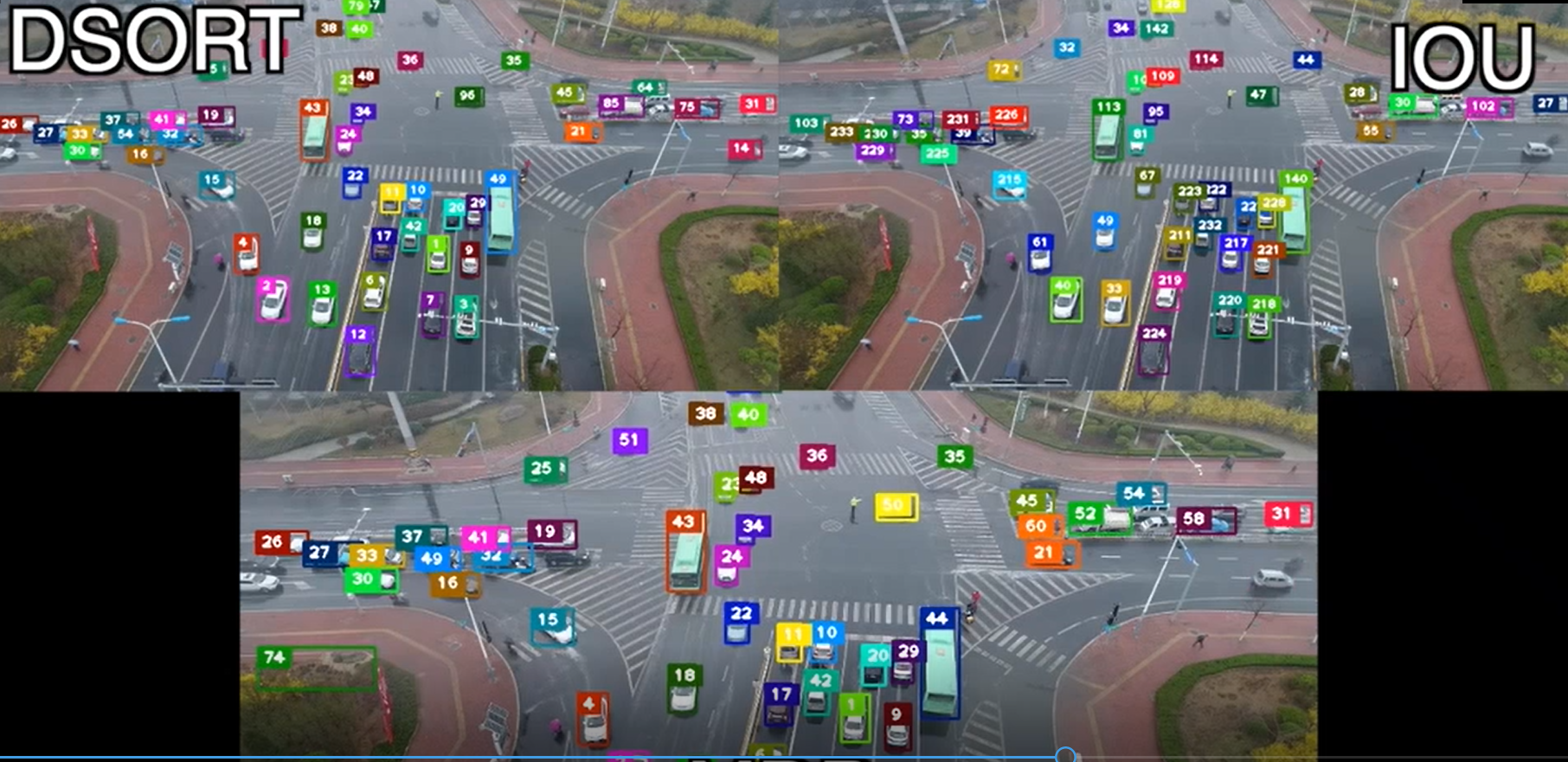## YOLO+Deepsort 全解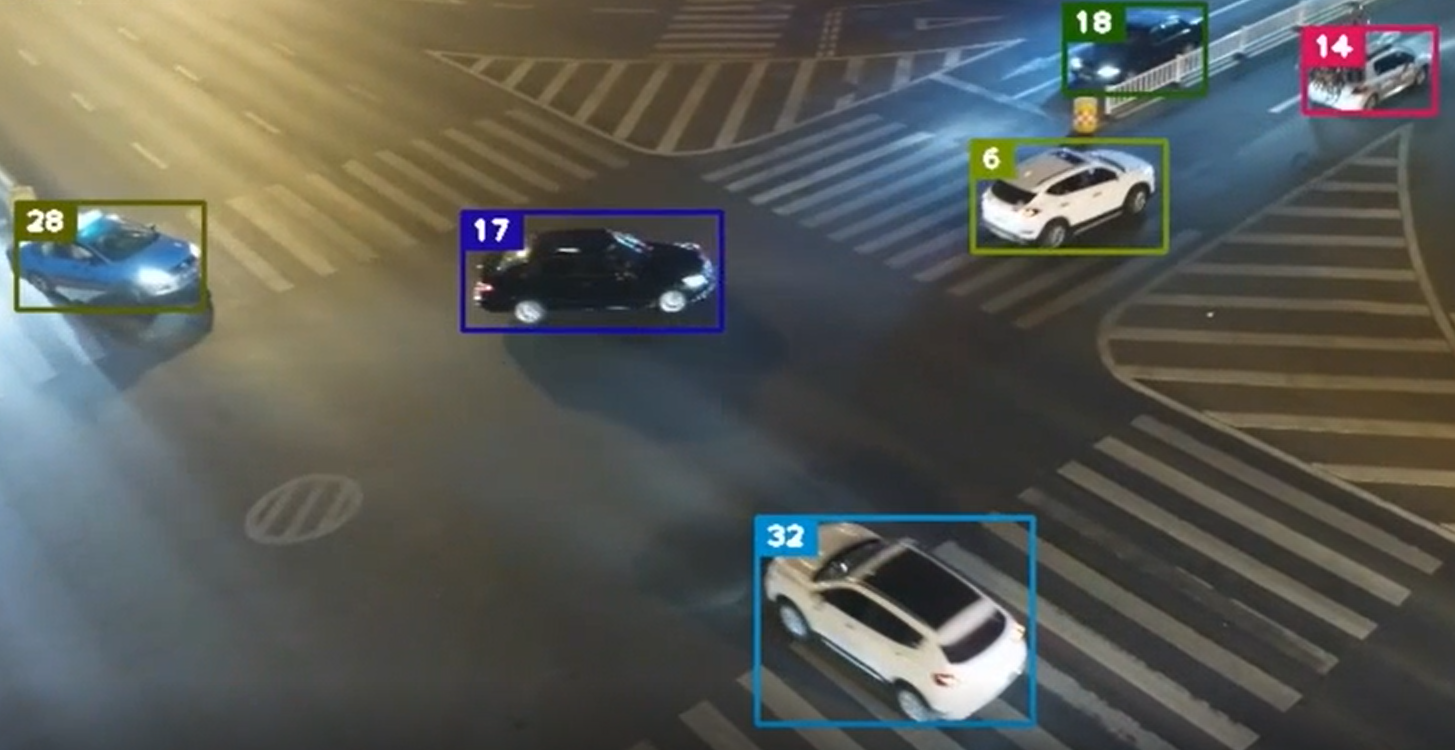## 浅析 SORT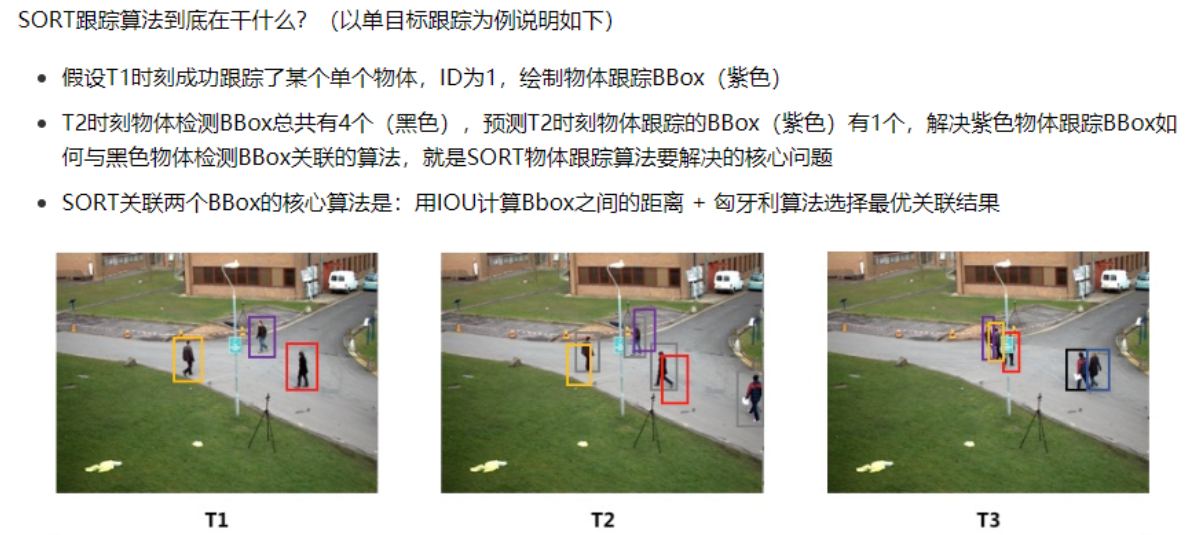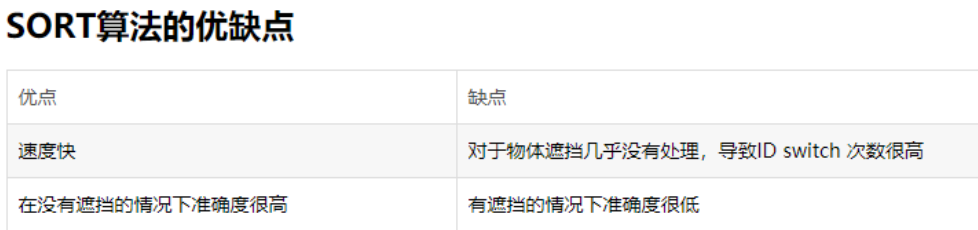### SORT 代码实现+解读：

from __future__ import print_function from numba import jit      #是python的一个JIT库，通过装饰器来实现运行时的加速import os.pathimport numpy as npimport matplotlib.pyplot as pltimport matplotlib.patches as patches  #用于绘制常见图像（如矩形，椭圆，圆形，多边形）from skimage import iofrom sklearn.utils.linear_assignment_ import linear_assignmentimport globimport timeimport argparsefrom filterpy.kalman import KalmanFilter  #filterpy包含了一些常用滤波器的库 @jit  #用了jit装饰器，可加速for循环的计算def iou(bb_test,bb_gt):  """  Computes IOU between two bboxes in the form [x1,y1,x2,y2]  """  xx1 = np.maximum(bb_test, bb_gt)  yy1 = np.maximum(bb_test, bb_gt)  xx2 = np.minimum(bb_test, bb_gt)  yy2 = np.minimum(bb_test, bb_gt)  w = np.maximum(0., xx2 - xx1)  h = np.maximum(0., yy2 - yy1)  wh = w * h  o = wh / ((bb_test-bb_test)*(bb_test-bb_test)   #IOU=（bb_test和bb_gt框相交部分面积）/(bb_test框面积+bb_gt框面积 - 两者相交面积)    + (bb_gt-bb_gt)*(bb_gt-bb_gt) - wh)  return(o) def convert_bbox_to_z(bbox): #将bbox由[x1,y1,x2,y2]形式转为 [框中心点x,框中心点y,框面积s,宽高比例r]^T  """  Takes a bounding box in the form [x1,y1,x2,y2] and returns z in the form    [x,y,s,r] where x,y is the centre of the box and s is the scale/area and r is    the aspect ratio  """  w = bbox-bbox  h = bbox-bbox  x = bbox+w/2.  y = bbox+h/2.  s = w*h    #scale is just area  r = w/float(h)  return np.array([x,y,s,r]).reshape((4,1))  #将数组转为4行一列形式，即[x,y,s,r]^T def convert_x_to_bbox(x,score=None): #将[x,y,s,r]形式的bbox，转为[x1,y1,x2,y2]形式  """  Takes a bounding box in the centre form [x,y,s,r] and returns it in the form    [x1,y1,x2,y2] where x1,y1 is the top left and x2,y2 is the bottom right  """  w = np.sqrt(x*x)  #w=sqrt(w*h * w/h)  h = x/w              #h=w*h/w  if(score==None): #如果检测框不带置信度    return np.array([x-w/2.,x-h/2.,x+w/2.,x+h/2.]).reshape((1,4))  #返回[x1,y1,x2,y2]  else:            #如果加测框带置信度    return np.array([x-w/2.,x-h/2.,x+w/2.,x+h/2.,score]).reshape((1,5)) #返回[x1,y1,x2,y2,score]  class KalmanBoxTracker(object):  """  This class represents the internel state of individual tracked objects observed as bbox.  """  count = 0  def __init__(self,bbox):    """    Initialises a tracker using initial bounding box.  使用初始边界框初始化跟踪器    """    #define constant velocity model                #定义匀速模型    self.kf = KalmanFilter(dim_x=7, dim_z=4)       #状态变量是7维， 观测值是4维的，按照需要的维度构建目标    self.kf.F = np.array([[1,0,0,0,1,0,0],[0,1,0,0,0,1,0],[0,0,1,0,0,0,1],[0,0,0,1,0,0,0],[0,0,0,0,1,0,0],[0,0,0,0,0,1,0],[0,0,0,0,0,0,1]])    self.kf.H = np.array([[1,0,0,0,0,0,0],[0,1,0,0,0,0,0],[0,0,1,0,0,0,0],[0,0,0,1,0,0,0]])     self.kf.R[2:,2:] *= 10.    self.kf.P[4:,4:] *= 1000. #give high uncertainty to the unobservable initial velocities 对未观测到的初始速度给出高的不确定性    self.kf.P *= 10.          # 默认定义的协方差矩阵是np.eye(dim_x)，将P中的数值与10， 1000相乘，赋值不确定性    self.kf.Q[-1,-1] *= 0.01    self.kf.Q[4:,4:] *= 0.01     self.kf.x[:4] = convert_bbox_to_z(bbox)  #将bbox转为 [x,y,s,r]^T形式，赋给状态变量X的前4位    self.time_since_update = 0    self.id = KalmanBoxTracker.count    KalmanBoxTracker.count += 1    self.history = []    self.hits = 0    self.hit_streak = 0    self.age = 0   def update(self,bbox):    """    Updates the state vector with observed bbox.    """    self.time_since_update = 0    self.history = []    self.hits += 1    self.hit_streak += 1    self.kf.update(convert_bbox_to_z(bbox))   def predict(self):    """    Advances the state vector and returns the predicted bounding box estimate.    """    if((self.kf.x+self.kf.x)<=0):      self.kf.x *= 0.0    self.kf.predict()    self.age += 1    if(self.time_since_update>0):      self.hit_streak = 0    self.time_since_update += 1    self.history.append(convert_x_to_bbox(self.kf.x))    return self.history[-1]   def get_state(self):    """    Returns the current bounding box estimate.    """    return convert_x_to_bbox(self.kf.x) def associate_detections_to_trackers(detections,trackers,iou_threshold = 0.3):  #用于将检测与跟踪进行关联  """  Assigns detections to tracked object (both represented as bounding boxes)  Returns 3 lists of matches, unmatched_detections and unmatched_trackers  """  if(len(trackers)==0):  #如果跟踪器为空    return np.empty((0,2),dtype=int), np.arange(len(detections)), np.empty((0,5),dtype=int)  iou_matrix = np.zeros((len(detections),len(trackers)),dtype=np.float32) # 检测器与跟踪器IOU矩阵   for d,det in enumerate(detections):    for t,trk in enumerate(trackers):      iou_matrix[d,t] = iou(det,trk)   #计算检测器与跟踪器的IOU并赋值给IOU矩阵对应位置  matched_indices = linear_assignment(-iou_matrix)    # 参考：https://blog.csdn.net/herr_kun/article/details/86509591    加上负号是因为linear_assignment求的是最小代价组合，而我们需要的是IOU最大的组合方式，所以取负号   unmatched_detections = []    #未匹配上的检测器  for d,det in enumerate(detections):    if(d not in matched_indices[:,0]):  #如果检测器中第d个检测结果不在匹配结果索引中，则d未匹配上      unmatched_detections.append(d)  unmatched_trackers = []      #未匹配上的跟踪器  for t,trk in enumerate(trackers):    if(t not in matched_indices[:,1]):  #如果跟踪器中第t个跟踪结果不在匹配结果索引中，则t未匹配上      unmatched_trackers.append(t)   #filter out matched with low IOU   过滤掉那些IOU较小的匹配对  matches = []  #存放过滤后的匹配结果  for m in matched_indices:   #遍历粗匹配结果    if(iou_matrix[m,m]<iou_threshold):   #m是检测器ID， m是跟踪器ID，如它们的IOU小于阈值则将它们视为未匹配成功      unmatched_detections.append(m)      unmatched_trackers.append(m)    else:      matches.append(m.reshape(1,2))          #将过滤后的匹配对维度变形成1x2形式  if(len(matches)==0):           #如果过滤后匹配结果为空，那么返回空的匹配结果    matches = np.empty((0,2),dtype=int)    else:                          #如果过滤后匹配结果非空，则按0轴方向继续添加匹配对    matches = np.concatenate(matches,axis=0)   return matches, np.array(unmatched_detections), np.array(unmatched_trackers)  #其中跟踪器数组是5列的（最后一列是ID）   class Sort(object):  def __init__(self,max_age=1,min_hits=3):    """    Sets key parameters for SORT    """    self.max_age = max_age    self.min_hits = min_hits    self.trackers = []    self.frame_count = 0   def update(self,dets):  #输入的是检测结果[x1,y1,x2,y2,score]形式    """    Params:      dets - a numpy array of detections in the format [[x1,y1,x2,y2,score],[x1,y1,x2,y2,score],...]    Requires: this method must be called once for each frame even with empty detections.   #每一帧都得调用一次，即便检测结果为空    Returns the a similar array, where the last column is the object ID.                    #返回相似的数组，最后一列是目标ID    NOTE: The number of objects returned may differ from the number of detections provided.  #返回的目标数量可能与提供的检测数量不同    """    self.frame_count += 1   #帧计数    #get predicted locations from existing trackers.    trks = np.zeros((len(self.trackers),5)) # 根据当前所有卡尔曼跟踪器的个数创建二维零矩阵，维度为：卡尔曼跟踪器ID个数x 5 (这5列内容为bbox与ID)    to_del = []                             #存放待删除    ret = []                                #存放最后返回的结果    for t,trk in enumerate(trks):      #循环遍历卡尔曼跟踪器列表      pos = self.trackers[t].predict()           #用卡尔曼跟踪器t 预测 对应物体在当前帧中的bbox      trk[:] = [pos, pos, pos, pos, 0]      if(np.any(np.isnan(pos))):                     #如果预测的bbox为空，那么将第t个卡尔曼跟踪器删除        to_del.append(t)    trks = np.ma.compress_rows(np.ma.masked_invalid(trks))  #将预测为空的卡尔曼跟踪器所在行删除，最后trks中存放的是上一帧中被跟踪的所有物体在当前帧中预测的非空bbox    for t in reversed(to_del): #对to_del数组进行倒序遍历      self.trackers.pop(t)   #从跟踪器中删除 to_del中的上一帧跟踪器ID    matched, unmatched_dets, unmatched_trks = associate_detections_to_trackers(dets,trks)  #对传入的检测结果 与 上一帧跟踪物体在当前帧中预测的结果做关联，返回匹配的目标矩阵matched, 新增目标的矩阵unmatched_dets, 离开画面的目标矩阵unmatched_trks     #update matched trackers with assigned detections    for t,trk in enumerate(self.trackers):    # 对卡尔曼跟踪器做遍历      if(t not in unmatched_trks):                   #如果上一帧中的t还在当前帧画面中（即不在当前预测的离开画面的矩阵unmatched_trks中）        d = matched[np.where(matched[:,1]==t),0]  #说明卡尔曼跟踪器t是关联成功的，在matched矩阵中找到与其关联的检测器d        trk.update(dets[d,:])                     #用关联的检测结果d来更新卡尔曼跟踪器（即用后验来更新先验）     #create and initialise new trackers for unmatched detections  #对于新增的未匹配的检测结果，创建并初始化跟踪器    for i in unmatched_dets:                  #新增目标        trk = KalmanBoxTracker(dets[i,:])     #将新增的未匹配的检测结果dets[i,:]传入KalmanBoxTracker        self.trackers.append(trk)             #将新创建和初始化的跟踪器trk 传入trackers    i = len(self.trackers)    for trk in reversed(self.trackers):       #对新的卡尔曼跟踪器集进行倒序遍历        d = trk.get_state()                #获取trk跟踪器的状态 [x1,y1,x2,y2]               if((trk.time_since_update < 1) and (trk.hit_streak >= self.min_hits or self.frame_count <= self.min_hits)):          ret.append(np.concatenate((d,[trk.id+1])).reshape(1,-1)) # +1 as MOT benchmark requires positive        i -= 1        #remove dead tracklet        if(trk.time_since_update > self.max_age):          self.trackers.pop(i)    if(len(ret)>0):      return np.concatenate(ret)    return np.empty((0,5))    def parse_args():    """Parse input arguments."""    parser = argparse.ArgumentParser(description='SORT demo')    parser.add_argument('--display', dest='display', help='Display online tracker output (slow) [False]',action='store_true')    args = parser.parse_args()    return args if __name__ == '__main__':  # all train  sequences = ['PETS09-S2L1','TUD-Campus','TUD-Stadtmitte','ETH-Bahnhof','ETH-Sunnyday','ETH-Pedcross2','KITTI-13','KITTI-17','ADL-Rundle-6','ADL-Rundle-8','Venice-2']  args = parse_args()  display = args.display  phase = 'train'  total_time = 0.0  total_frames = 0  colours = np.random.rand(32,3) #used only for display  if(display):    if not os.path.exists('mot_benchmark'):      print('\n\tERROR: mot_benchmark link not found!\n\n    Create a symbolic link to the MOT benchmark\n    (https://motchallenge.net/data/2D_MOT_2015/#download). E.g.:\n\n    \$ ln -s /path/to/MOT2015_challenge/2DMOT2015 mot_benchmark\n\n')      exit()    plt.ion()  #用于动态绘制显示图像    fig = plt.figure()     if not os.path.exists('output'):    os.makedirs('output')    for seq in sequences:    mot_tracker = Sort() #create instance of the SORT tracker    创建Sort 跟踪实例    seq_dets = np.loadtxt('data/%s/det.txt'%(seq),delimiter=',') #load detections    #加载检测结果    with open('output/%s.txt'%(seq),'w') as out_file:      print("Processing %s."%(seq))      for frame in range(int(seq_dets[:,0].max())):   #确定视频序列总帧数，并进行for循环        frame += 1 #detection and frame numbers begin at 1  #由于视频序列帧数是从1开始的，因此加1        dets = seq_dets[seq_dets[:,0]==frame,2:7]     #提取检测结果中的[x1,y1,w,h,score]到dets        dets[:,2:4] += dets[:,0:2] #convert to [x1,y1,w,h] to [x1,y1,x2,y2]   将dets中的第2,3列的数加上第0,1列的数后赋值给2,3列；        total_frames += 1          #总帧数累计         if(display):        #如果要求显示结果          ax1 = fig.add_subplot(111, aspect='equal')          fn = 'mot_benchmark/%s/%s/img1/%06d.jpg'%(phase,seq,frame)   #原图像路径名          im =io.imread(fn)      #加载图像          ax1.imshow(im)         #显示图像          plt.title(seq+' Tracked Targets')         start_time = time.time()        trackers = mot_tracker.update(dets)  #sort跟踪器更新        cycle_time = time.time() - start_time  #sort跟踪器耗时        total_time += cycle_time               #sort跟踪器总共耗费时间         for d in trackers:          print('%d,%d,%.2f,%.2f,%.2f,%.2f,1,-1,-1,-1'%(frame,d,d,d,d-d,d-d),file=out_file) #打印： frame,ID,x1,y1,x2,y2,1,-1,-1,-1          if(display):             #如果显示，将目标检测框画上            d = d.astype(np.int32)            ax1.add_patch(patches.Rectangle((d,d),d-d,d-d,fill=False,lw=3,ec=colours[d%32,:]))            ax1.set_adjustable('box-forced')         if(display):          fig.canvas.flush_events()          plt.draw()          ax1.cla()   print("Total Tracking took: %.3f for %d frames or %.1f FPS"%(total_time,total_frames,total_frames/total_time))  if(display):    print("Note: to get real runtime results run without the option: --display")

## DeepSORT 深入解读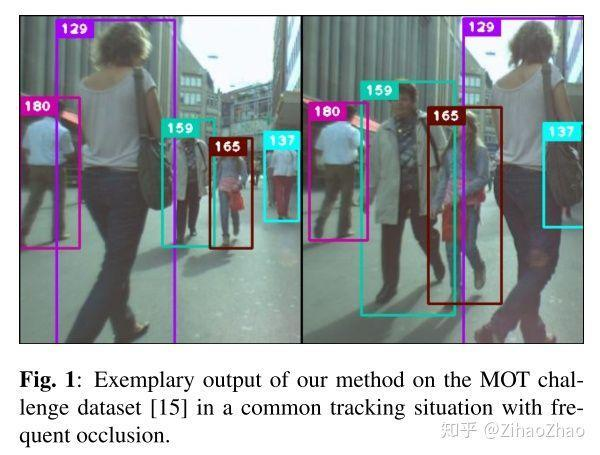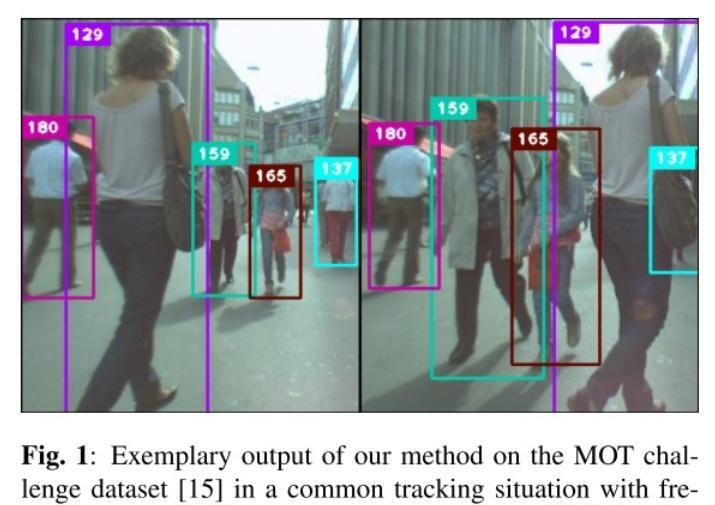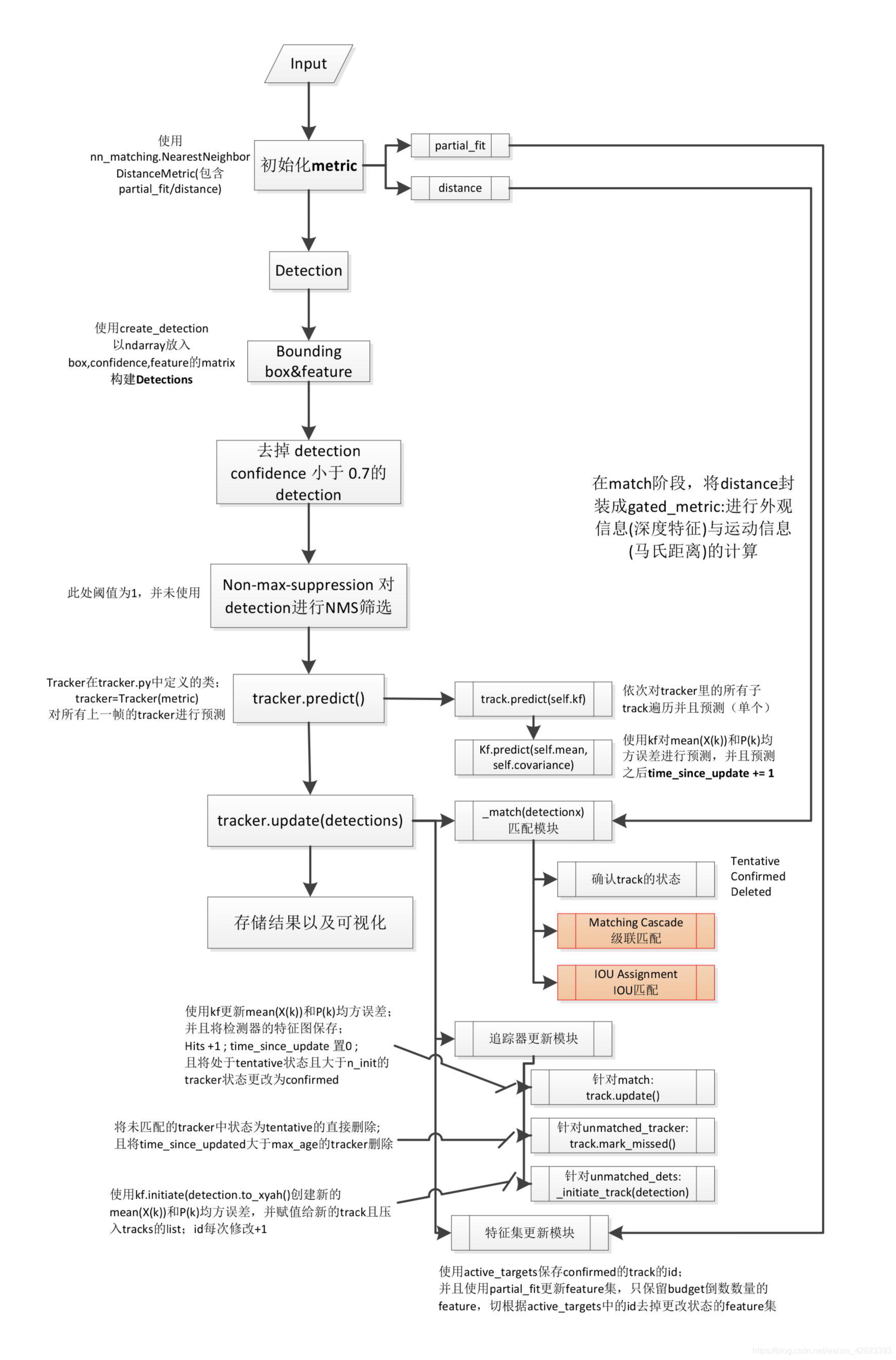IOU 匹配流程图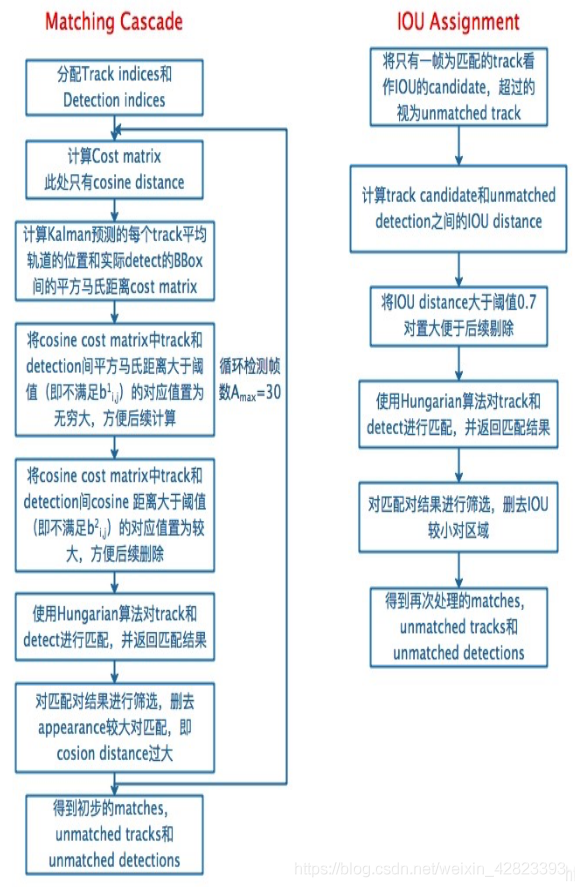## yolo 的总体思想归纳：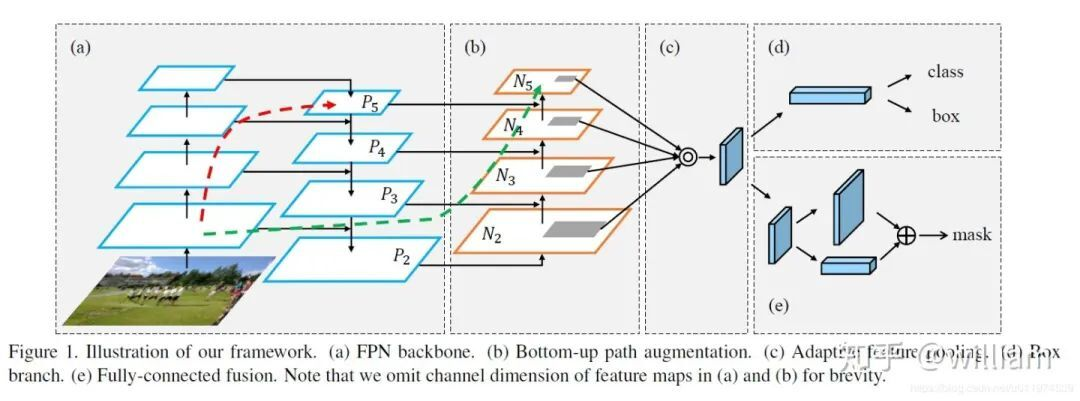​ csp 一个特征网络

YOLOv3 和 YOLOv5 整体思想类似，不过 YOLOV5 增加了 PaNet 等 tricks，以及 Mossiac 等大量数据增强，

yov5 使用 giou loss 作为边界框的损失。

yolov5 使用二元交叉熵和 Logits 损失函数来计算类损失概率和目标得分。我们也可以用 fl_uγ参数激活焦损来计算损失函数。 在 yolov5 中，中间/隐藏层采用漏 relu 激活函数，最终检测层采用 sigmoid 激活函数。

### YOLOv3/YOLOv5 训练教程：

2：数据标注与预处理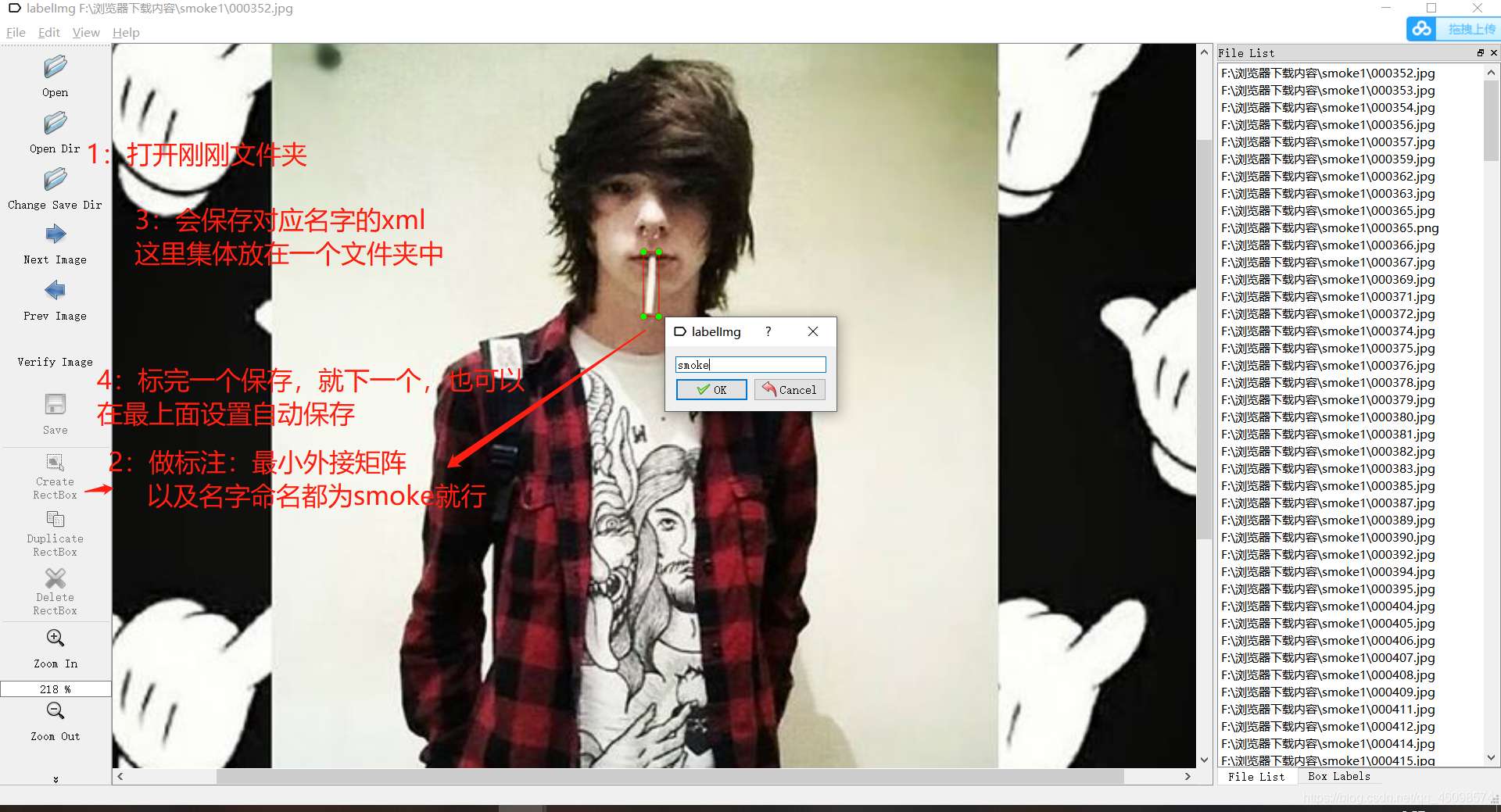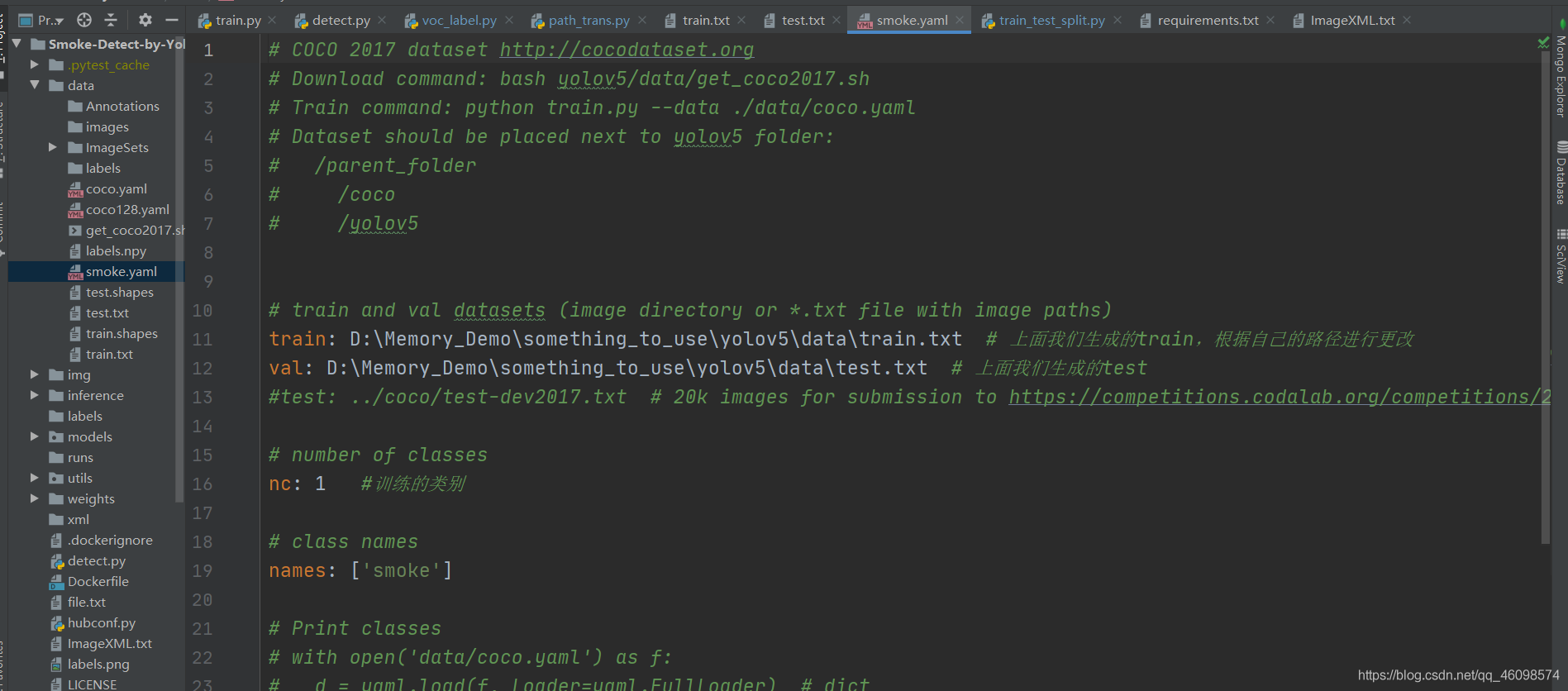python train.py --data data/smoke.yaml --cfg models/yolov5s.yaml --weights weights/yolov5s.pt --batch-size 10 --epochs 100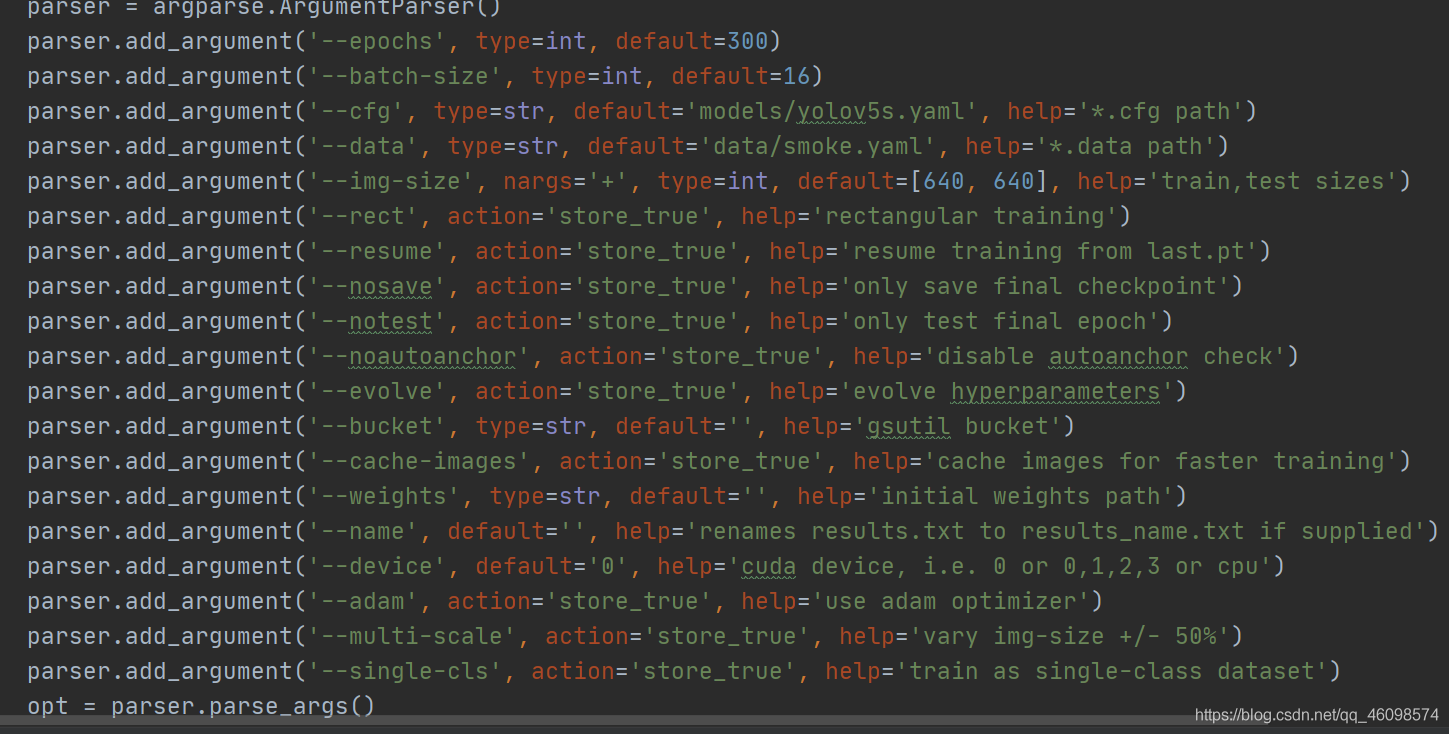We–data/smoke.yaml 是用 smoke.yaml 编写的训练代码路径和类别数据，通过它我们可以得到训练图片和标签。 然后使用-CFG models/yolov5s.yaml 和--weights/yolov5s.pt 获得配置模型和预训练模型的权重 批量大小 10 大家都知道，默认是 16，可以改成 16。在 yolov5s 中，模型参数不多，大约一百万，所以视频内存的消耗并不多。我的配置很差。使用 yolov5m 时，视频存储器 4G 不能调整为 16。 如果可以报告 CUDA 内存不足错误，只需减小批处理大小。 最后，epoch，我不需要解释，我用 yolov5m 训练了 24 小时 5 公里的图片，用 100 epoch 训练了 13 分钟。 在训练过程中，在跑步过程中会缓慢产生张力板，减少视觉损失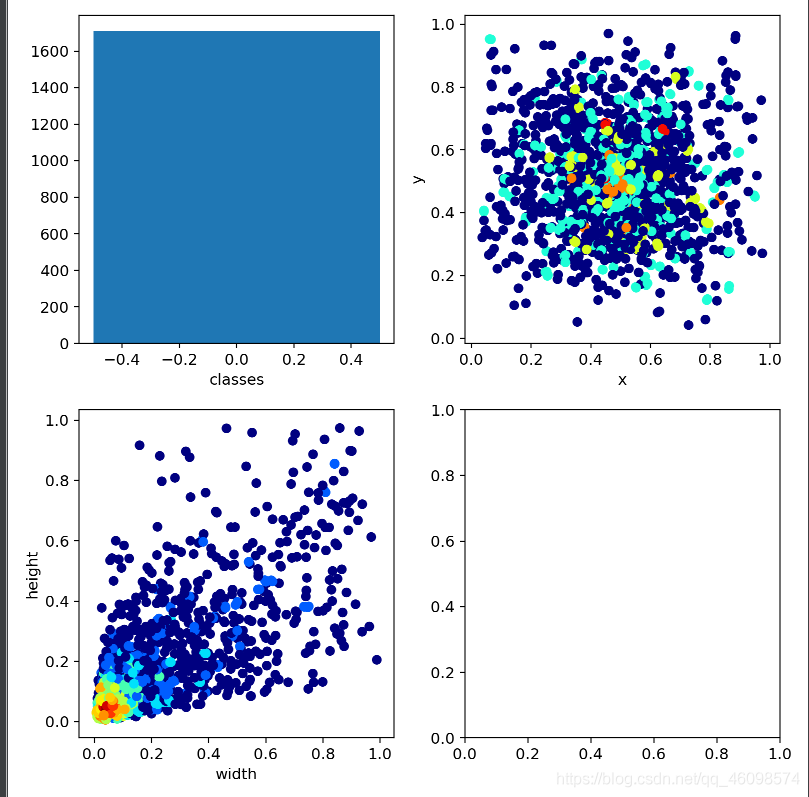### 检测：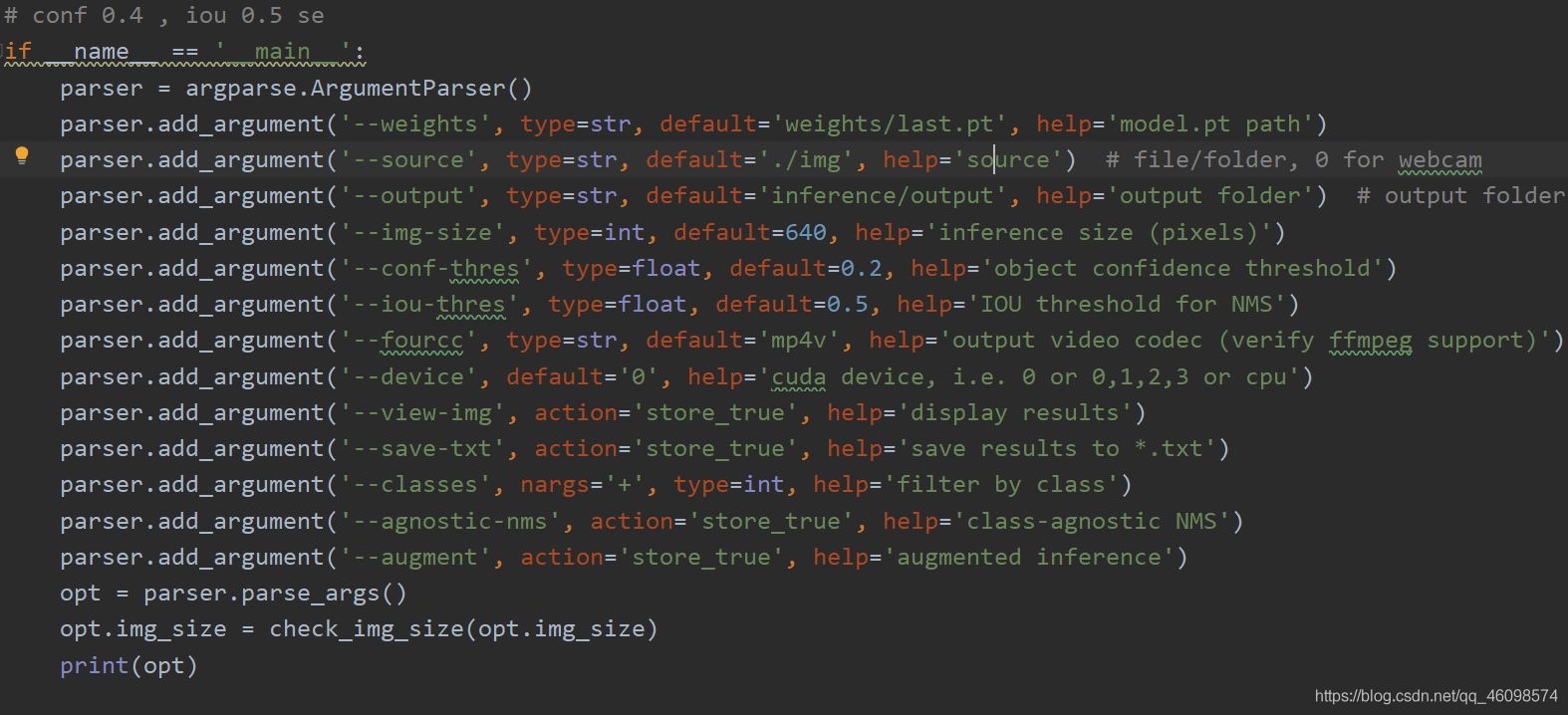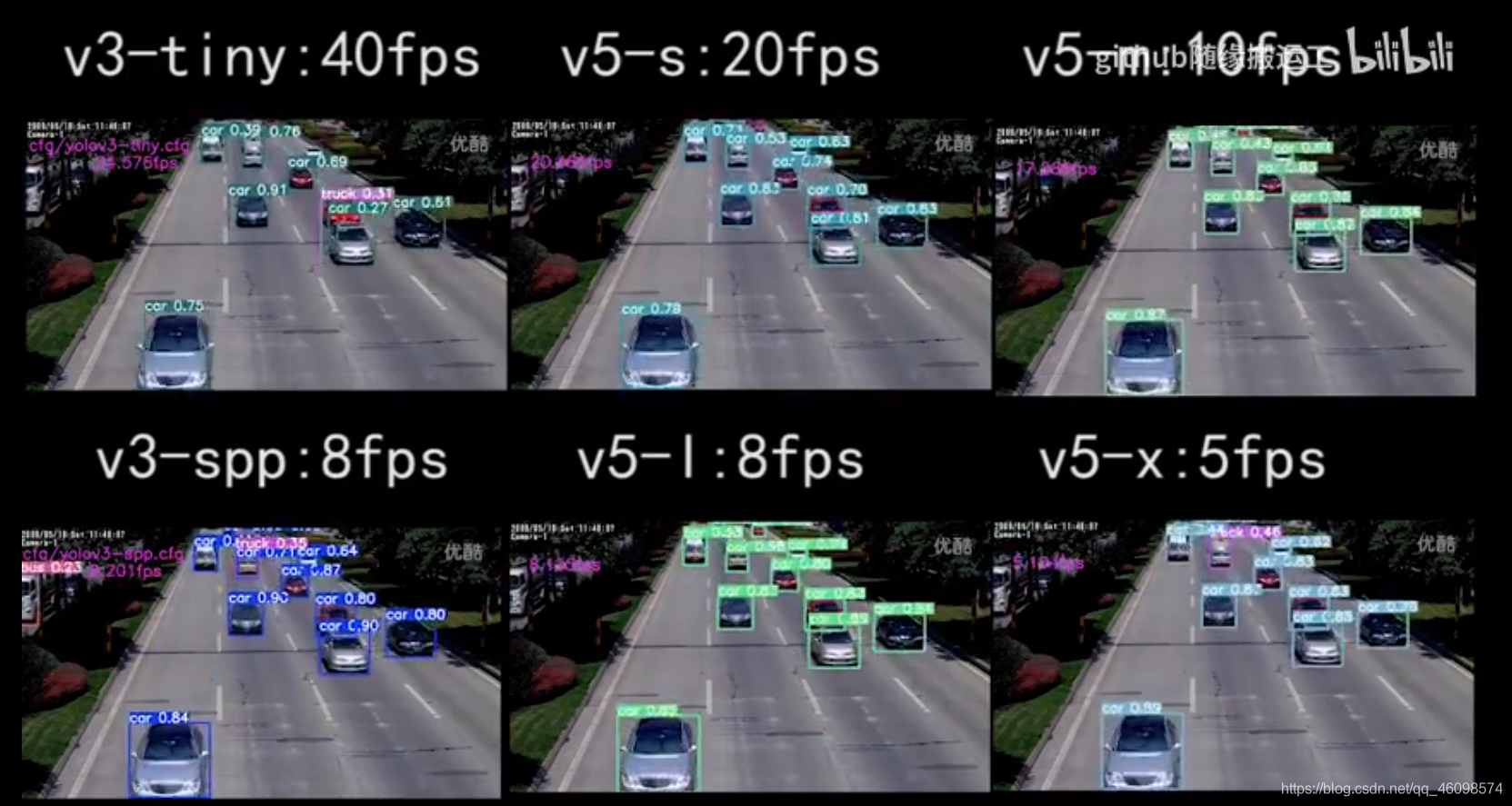## 代码介绍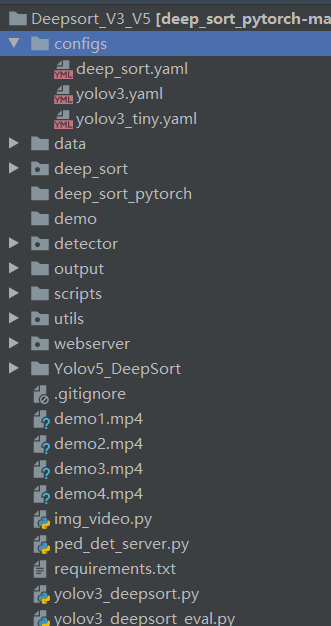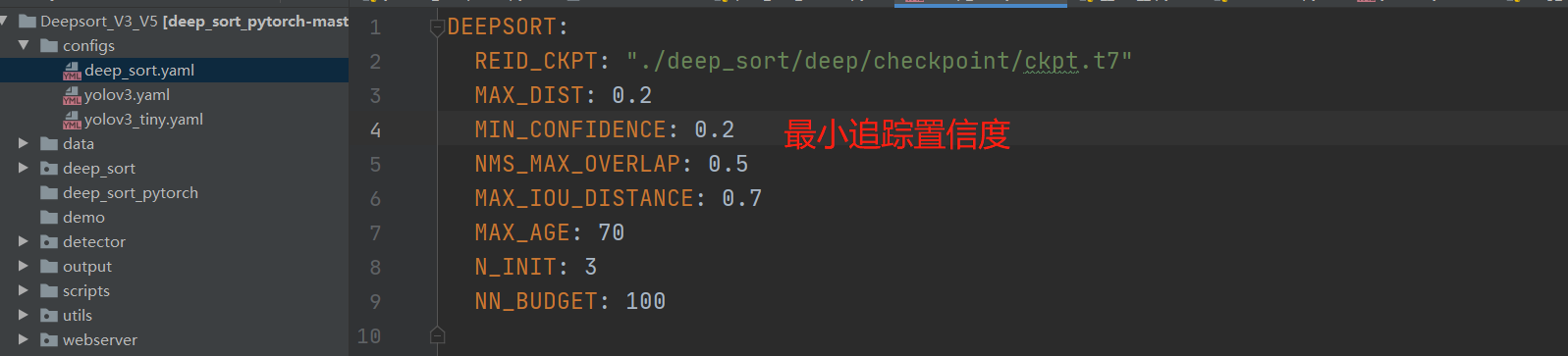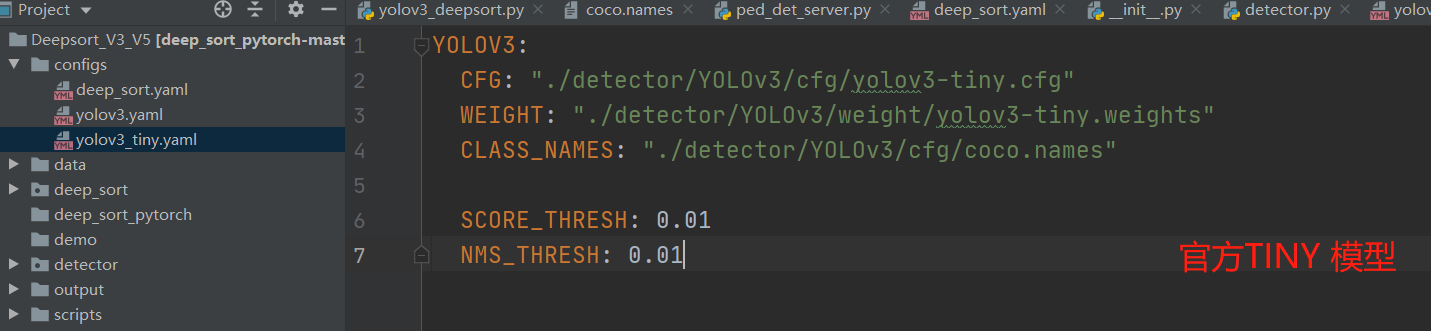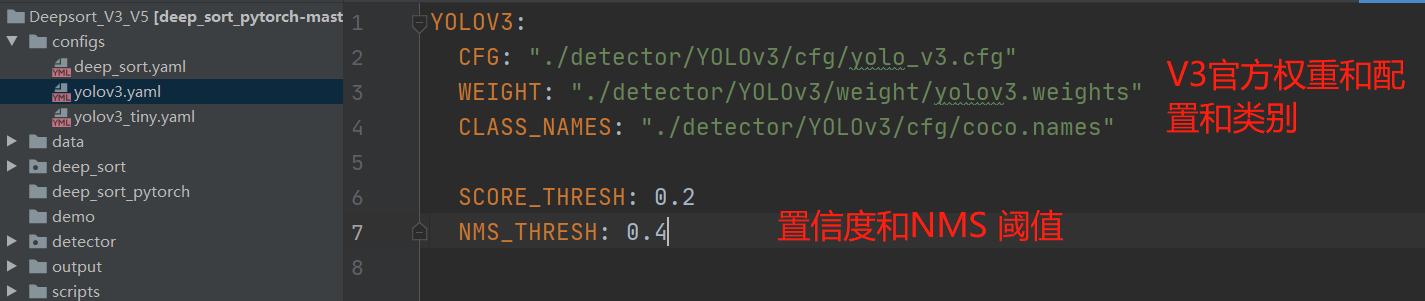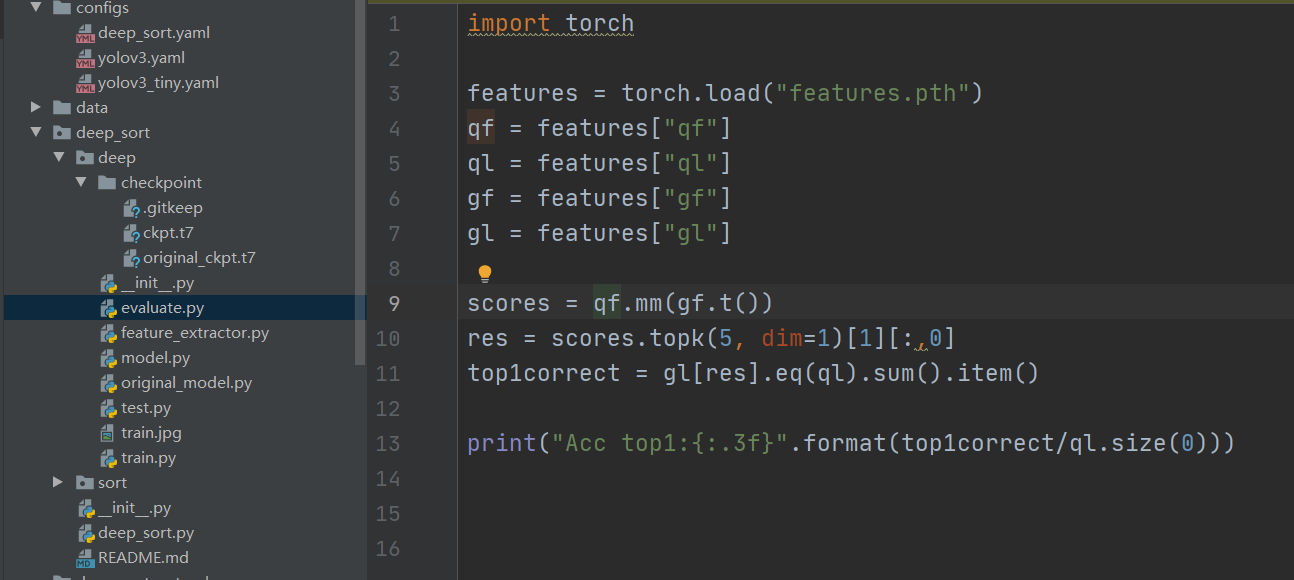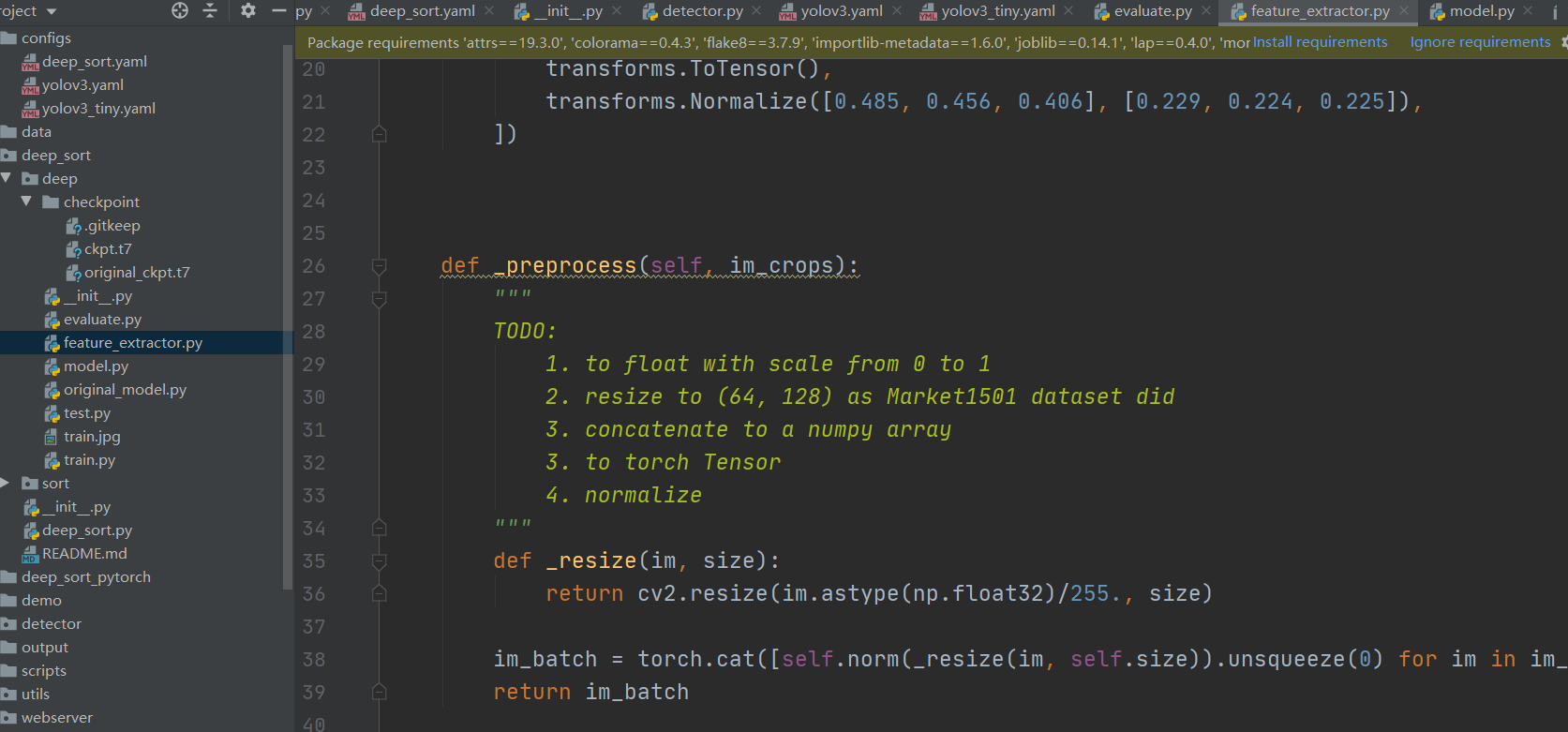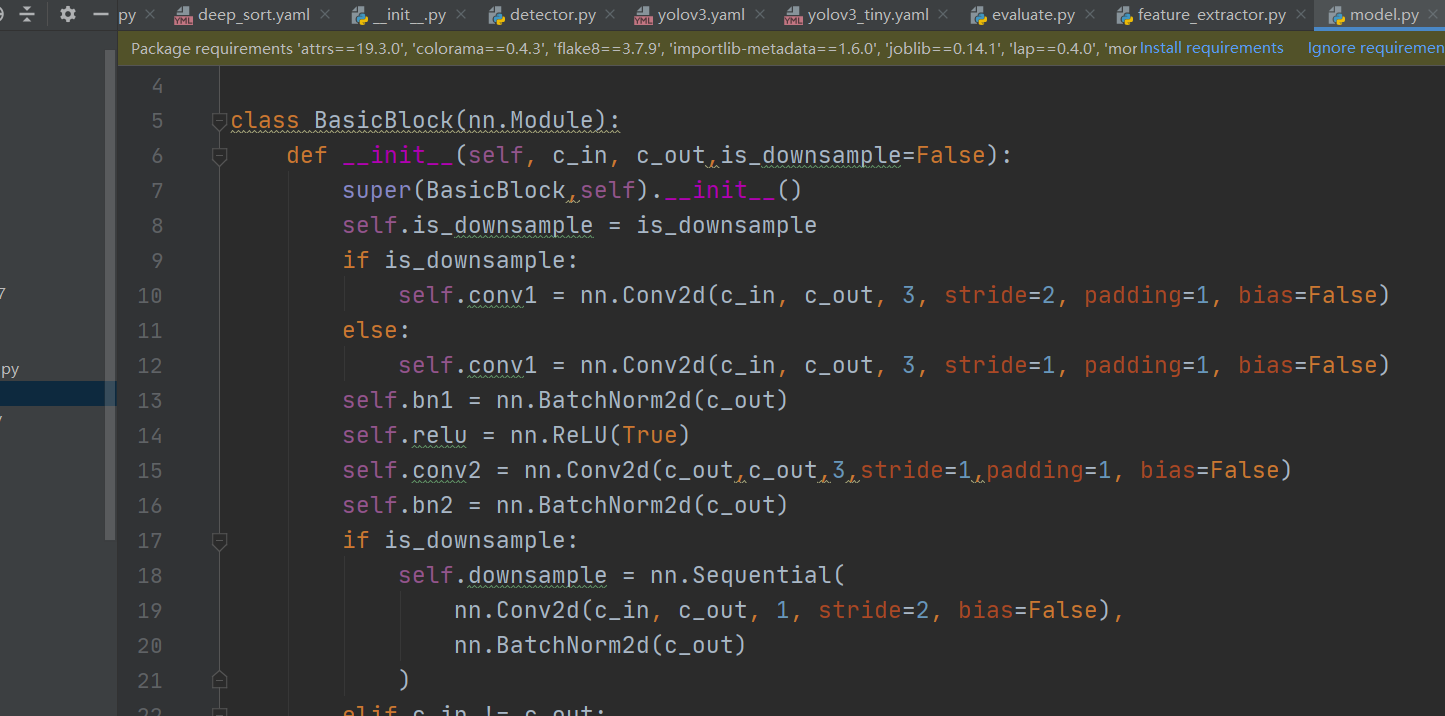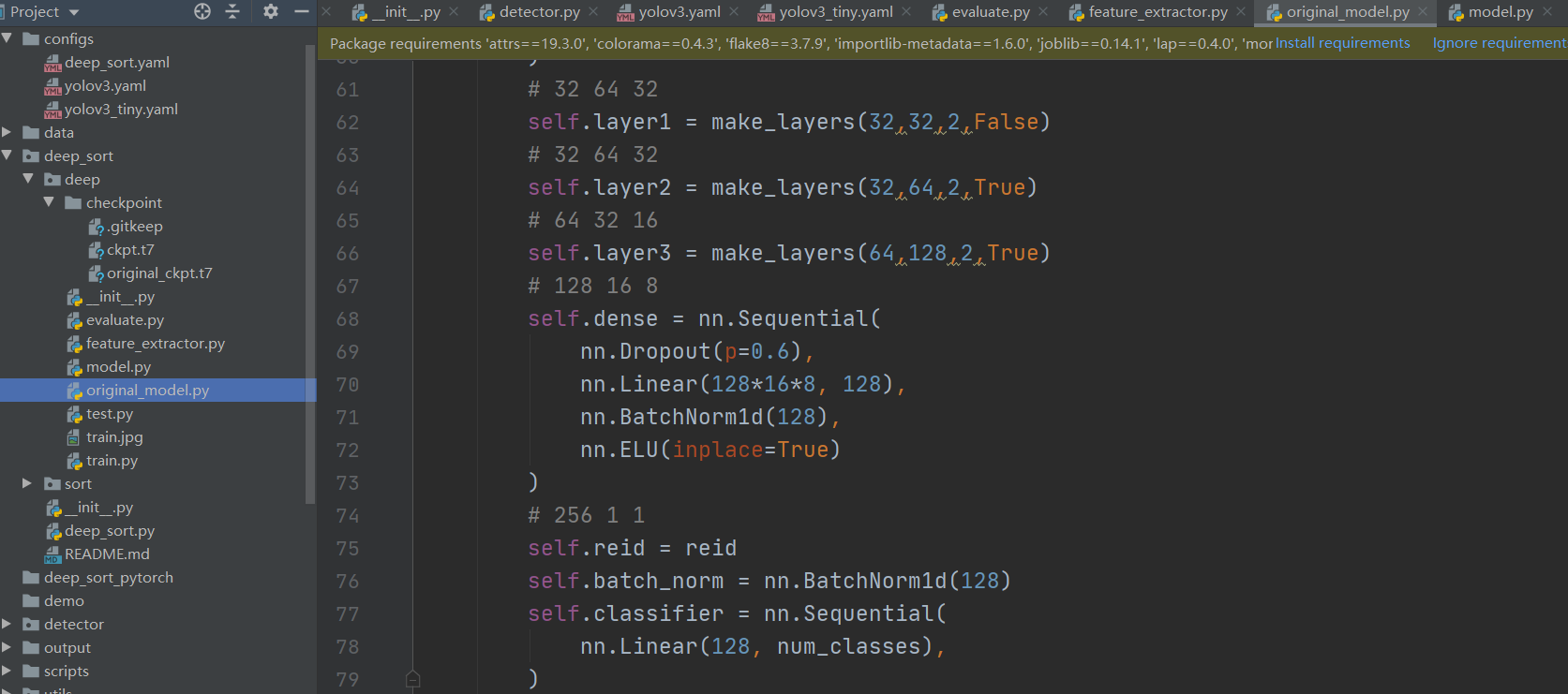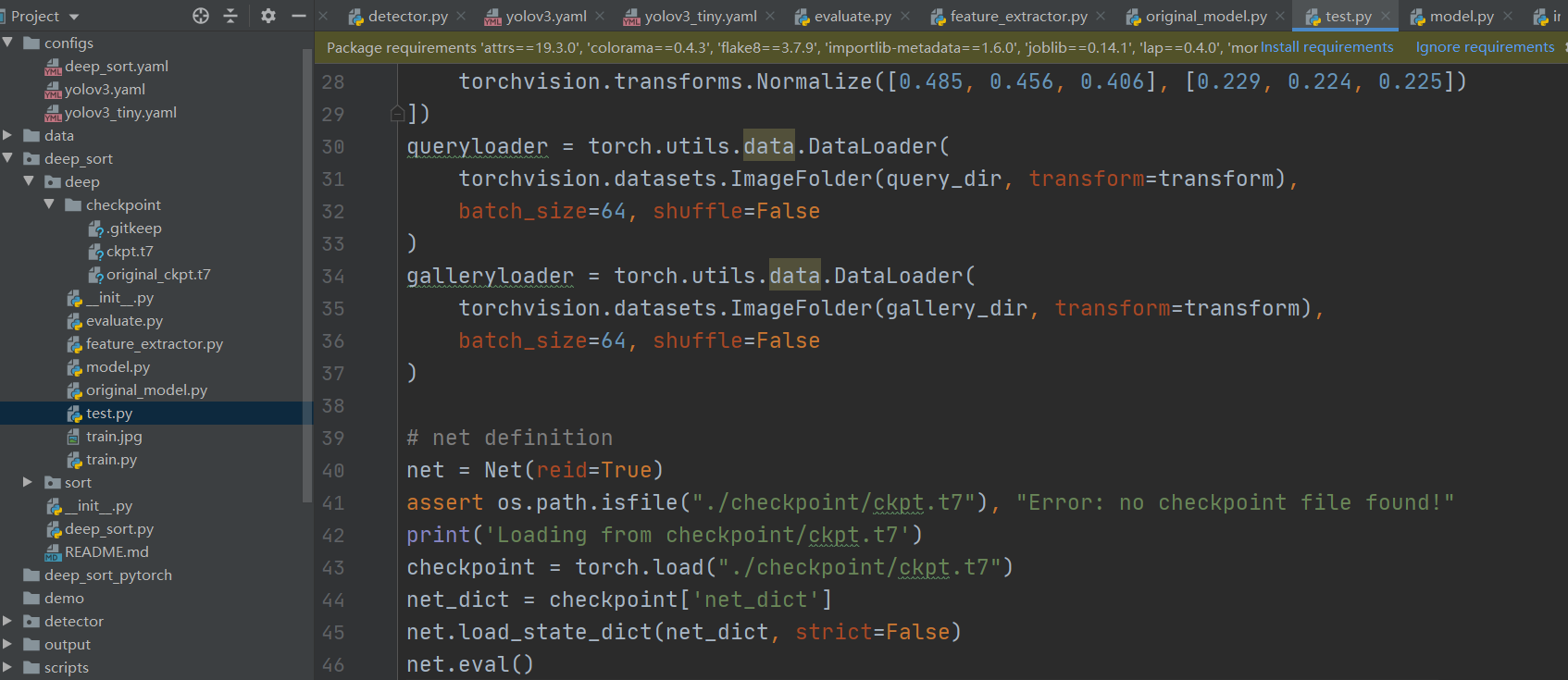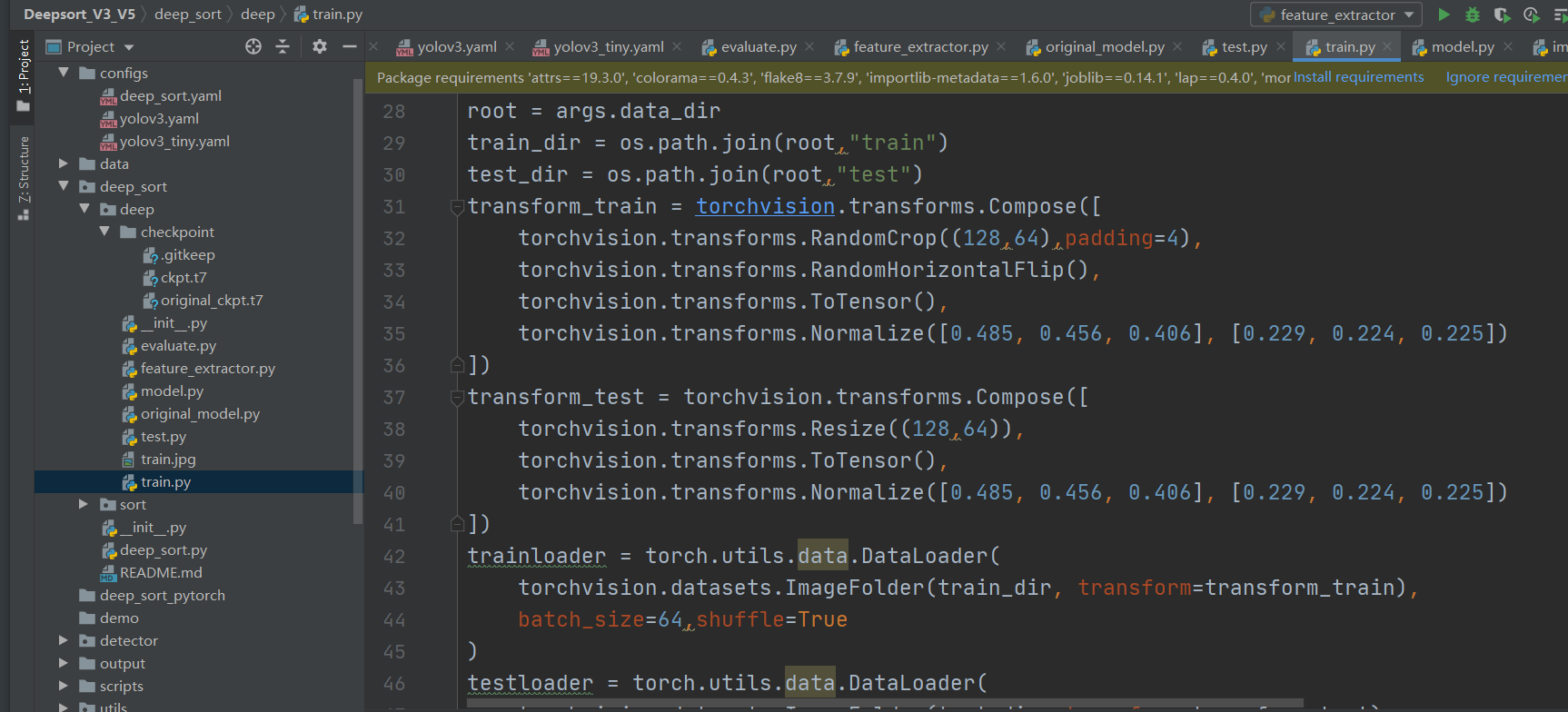### 追踪部分：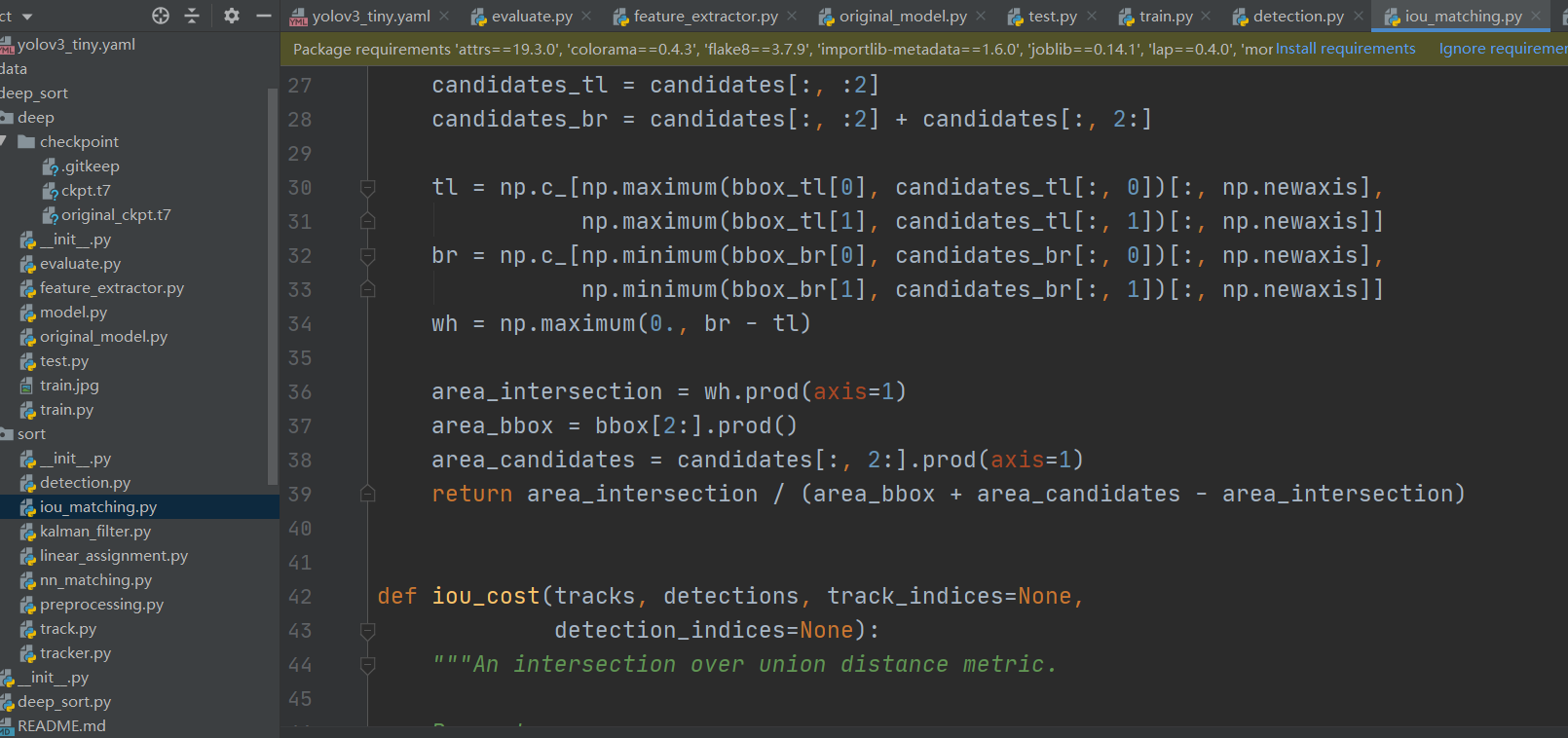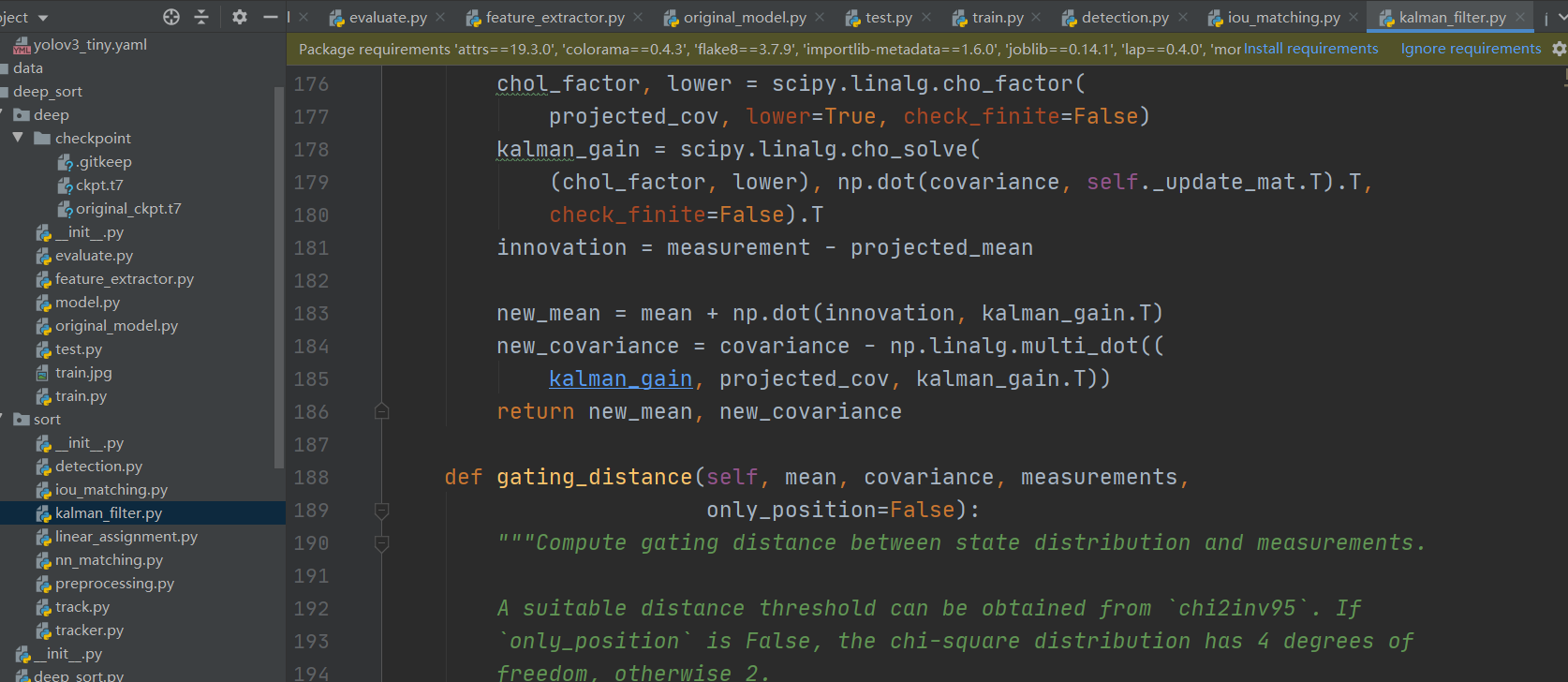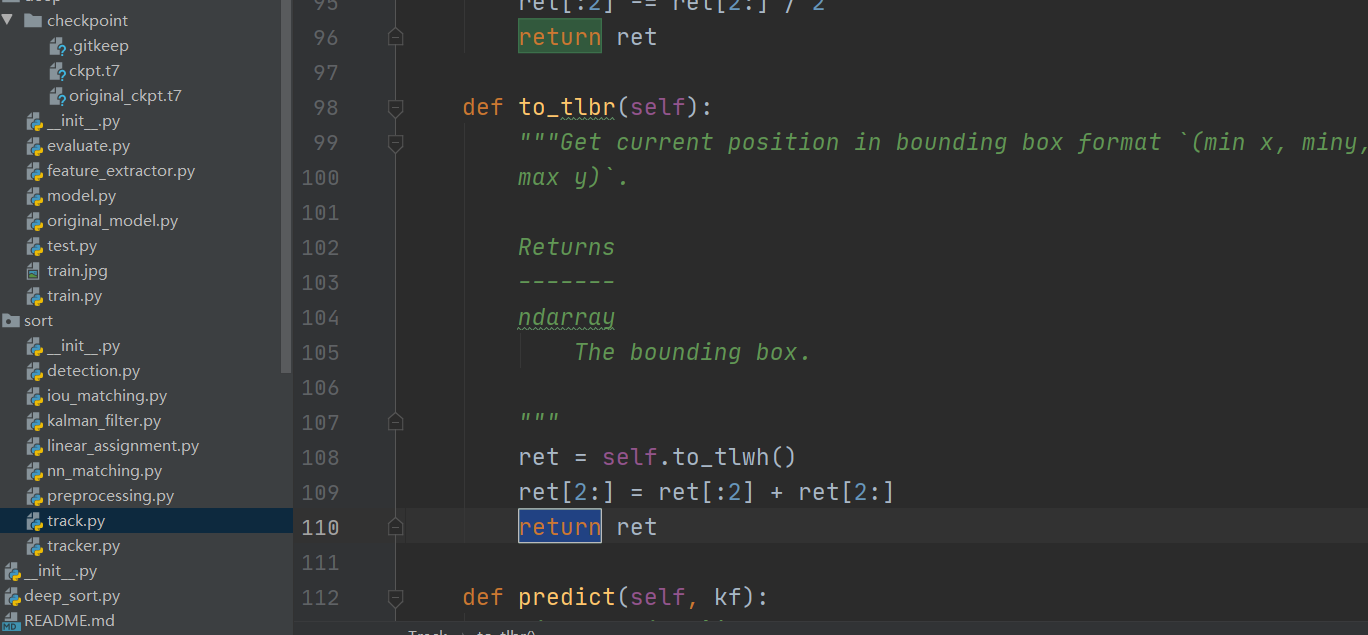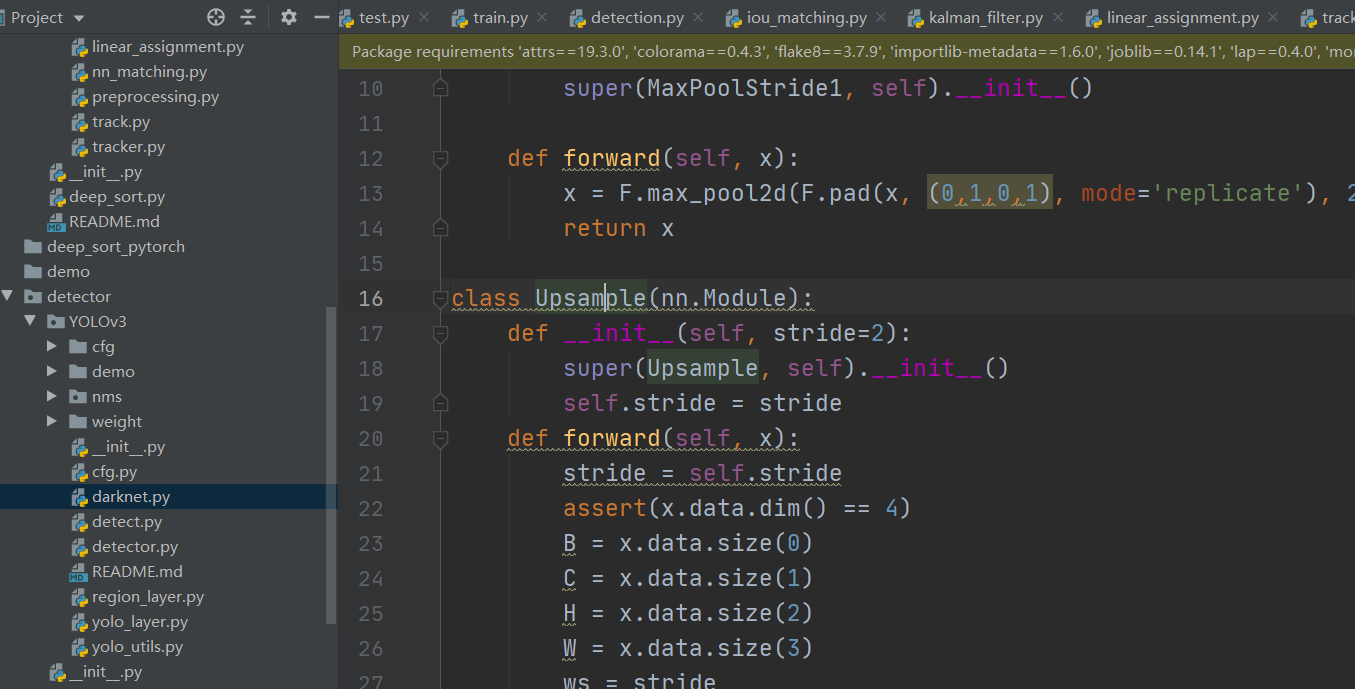### YOLOv3 部分，darknet 版本，的 pytorch 版。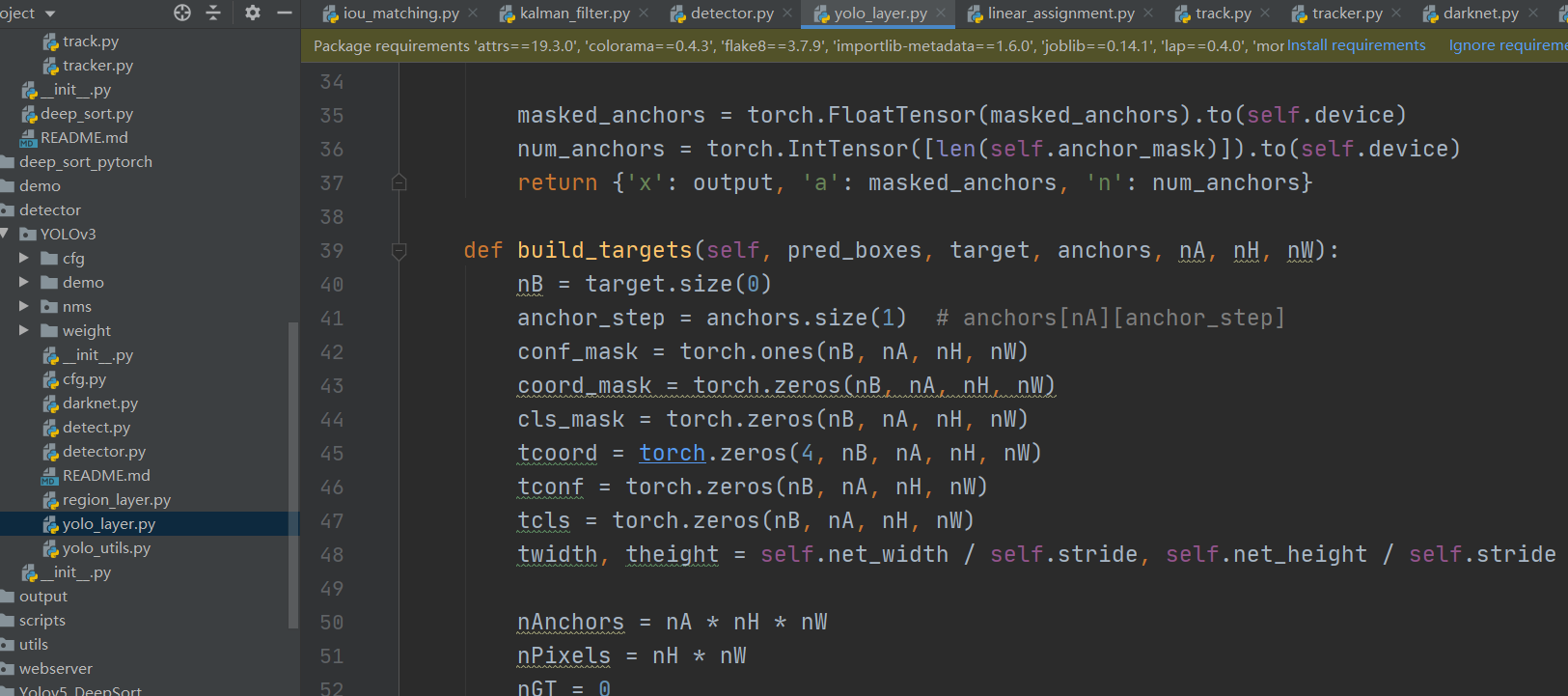YOLOv3 网络层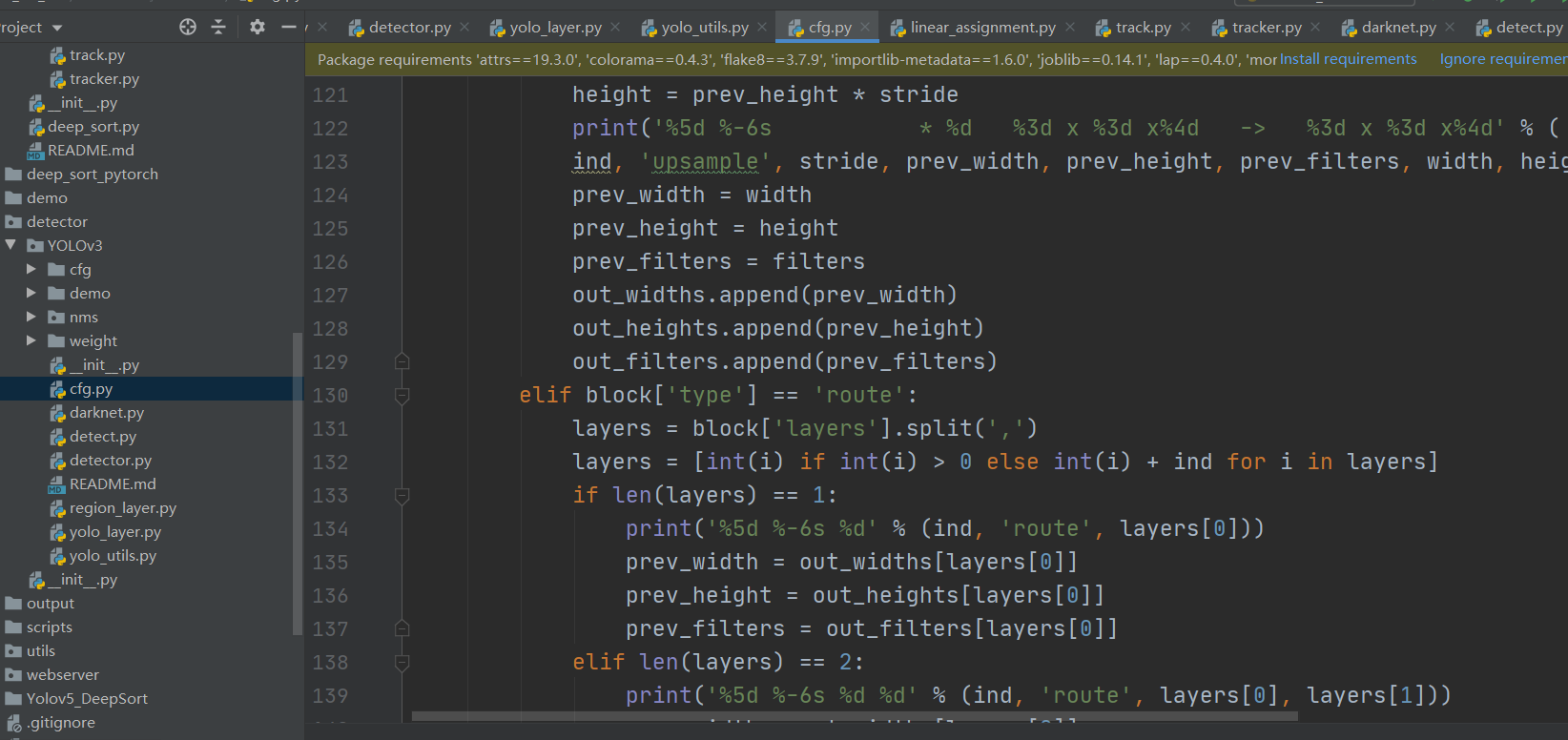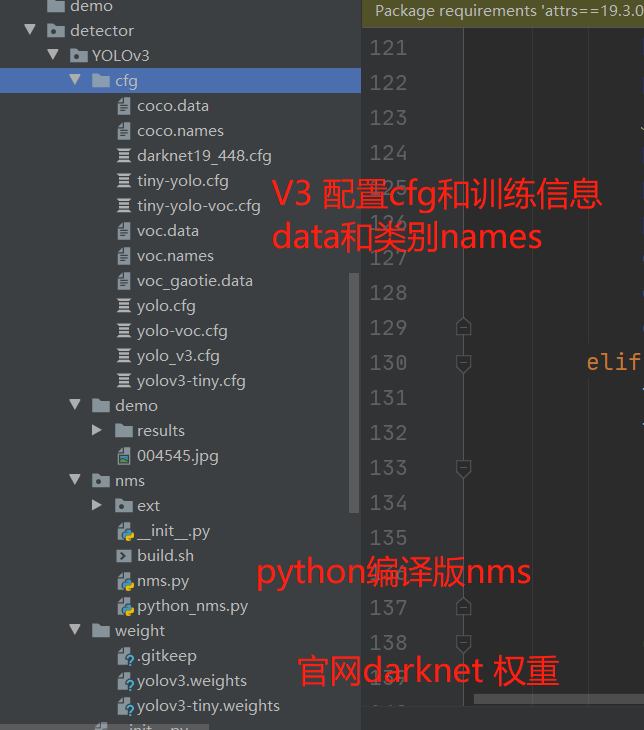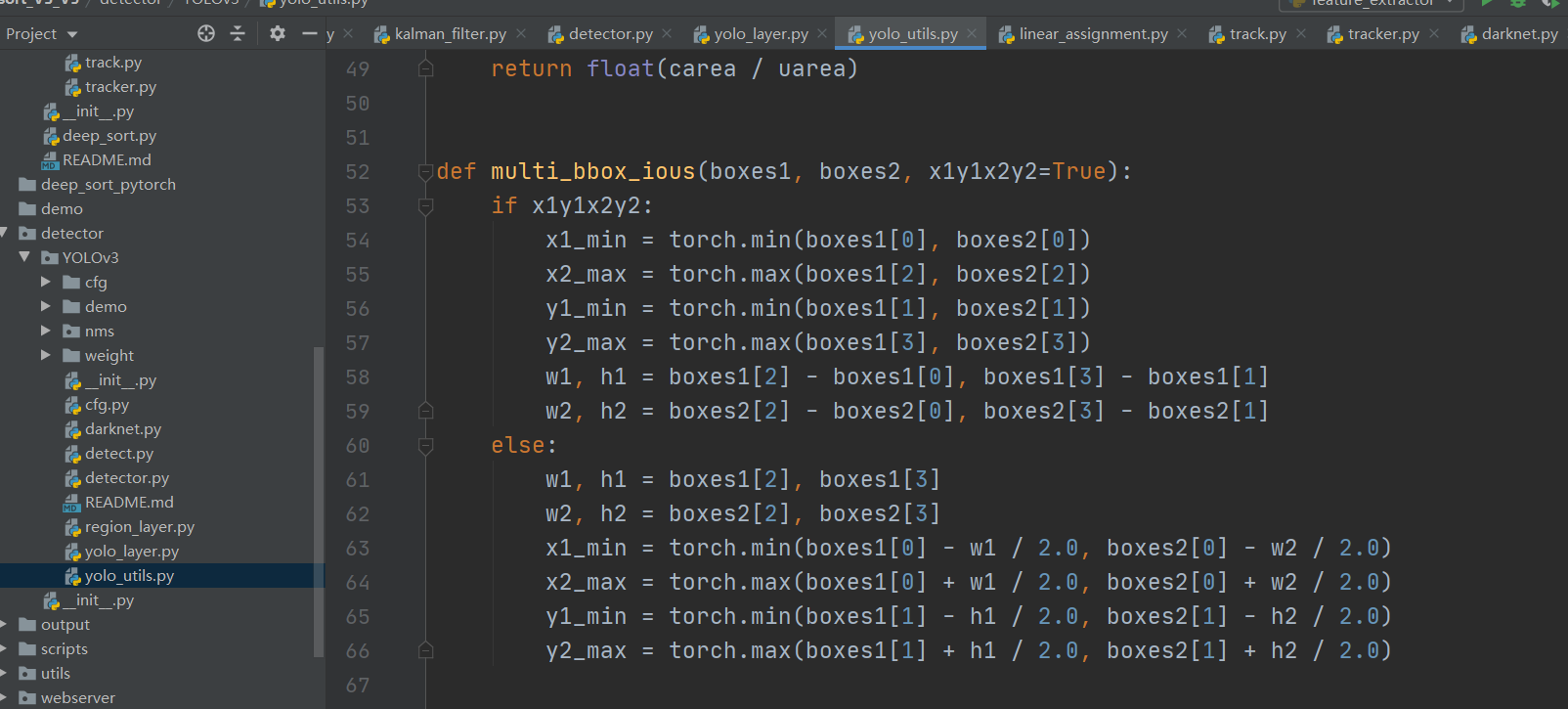Rtsp 推流等方式 web 端部署。

### V5 区域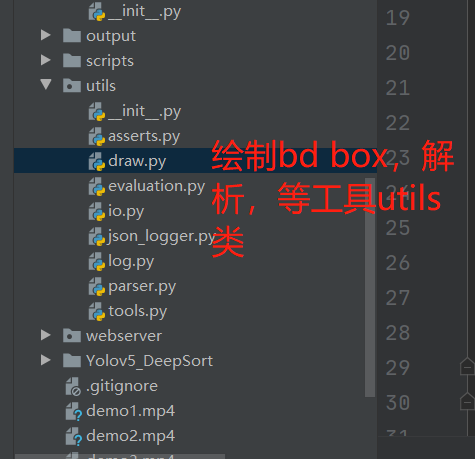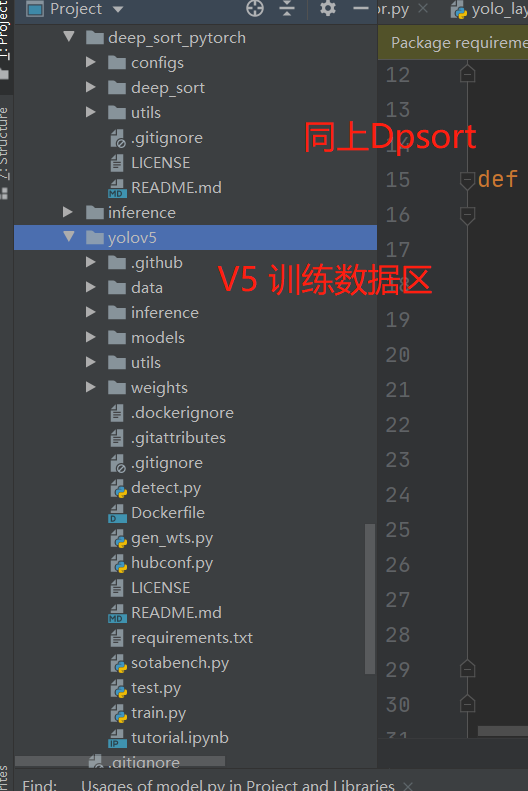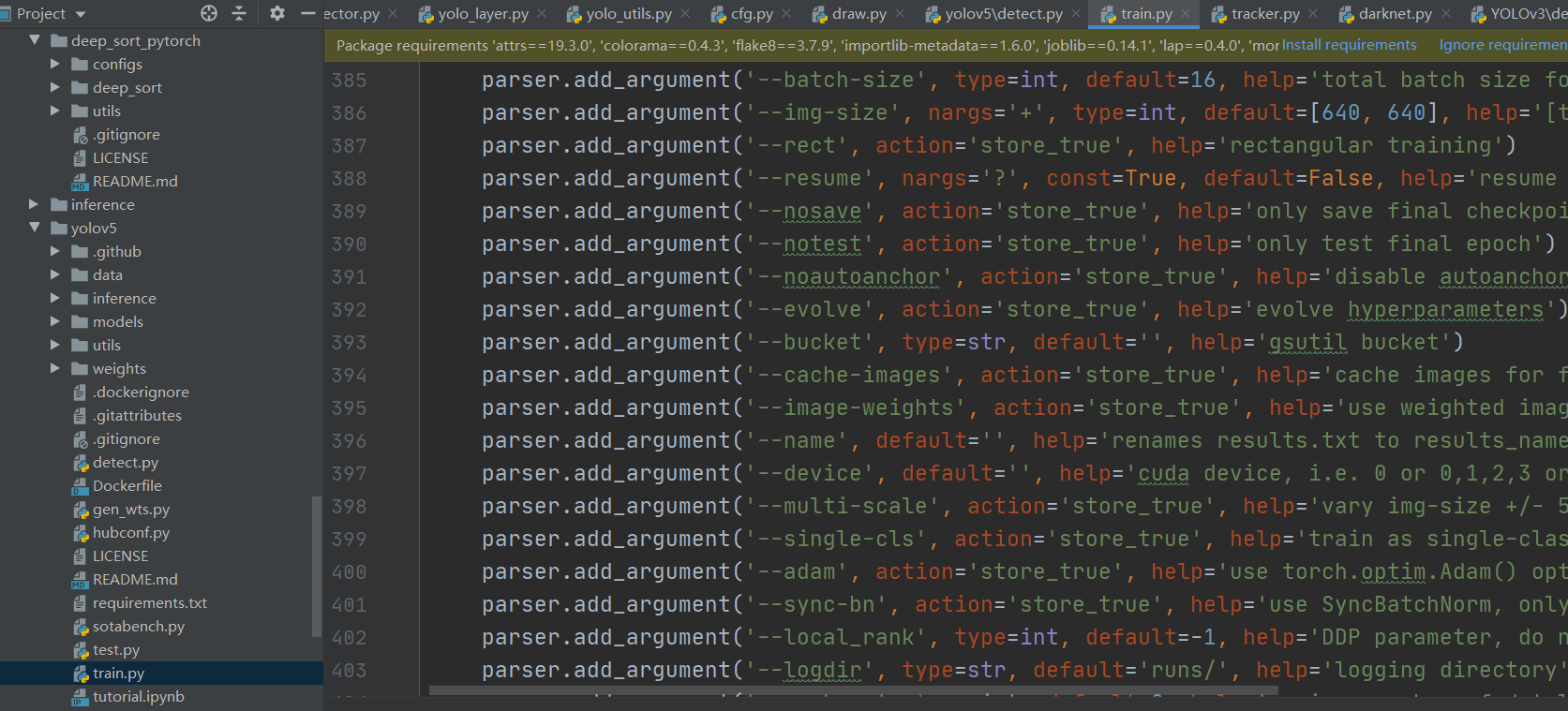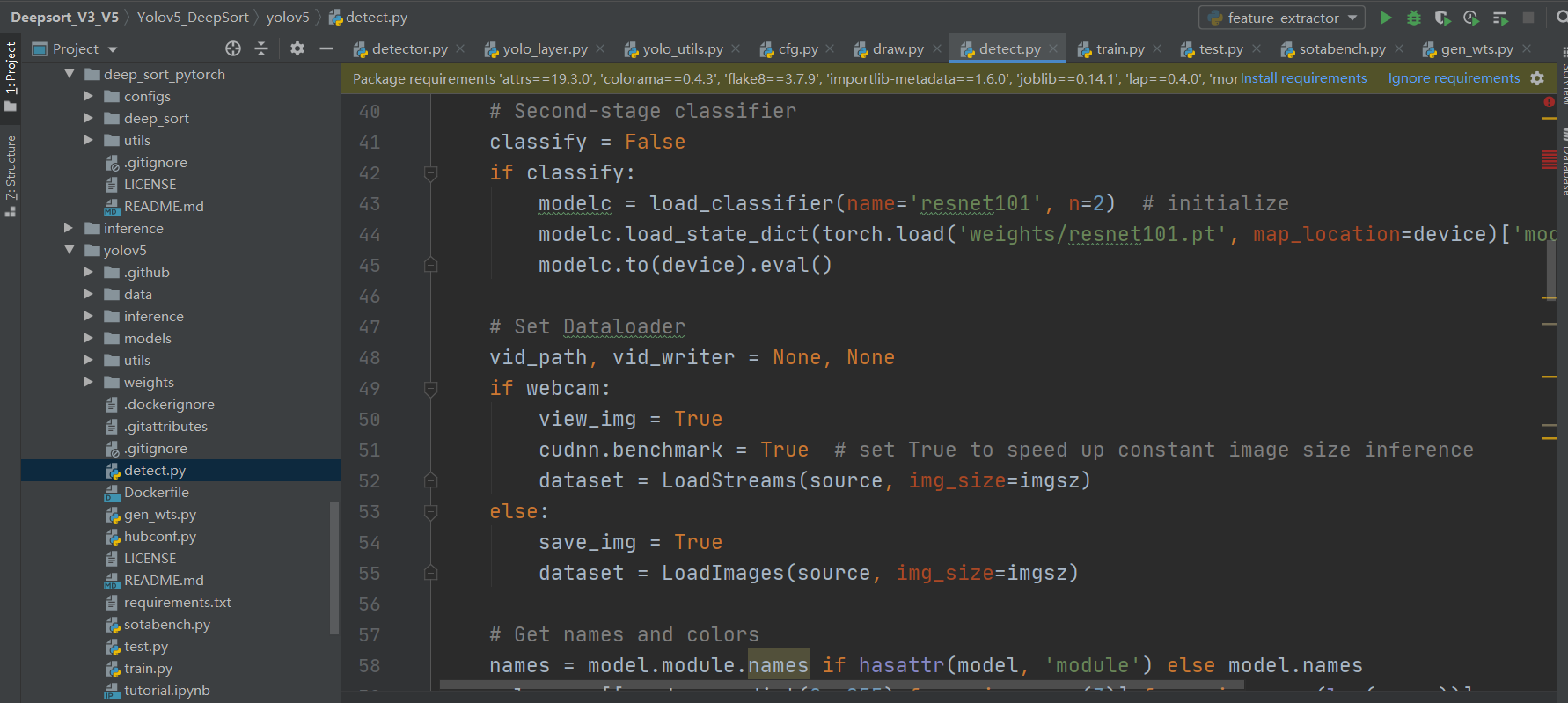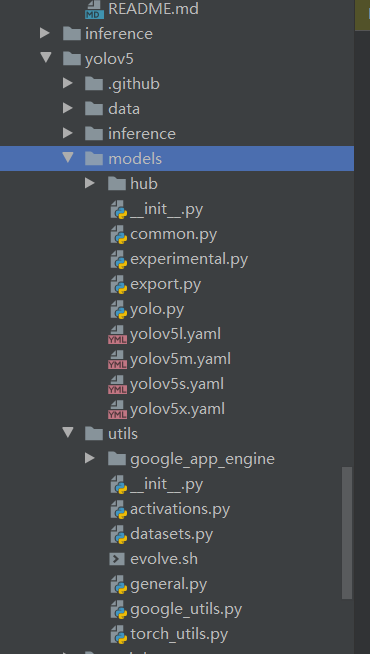V5 导出模型 onnx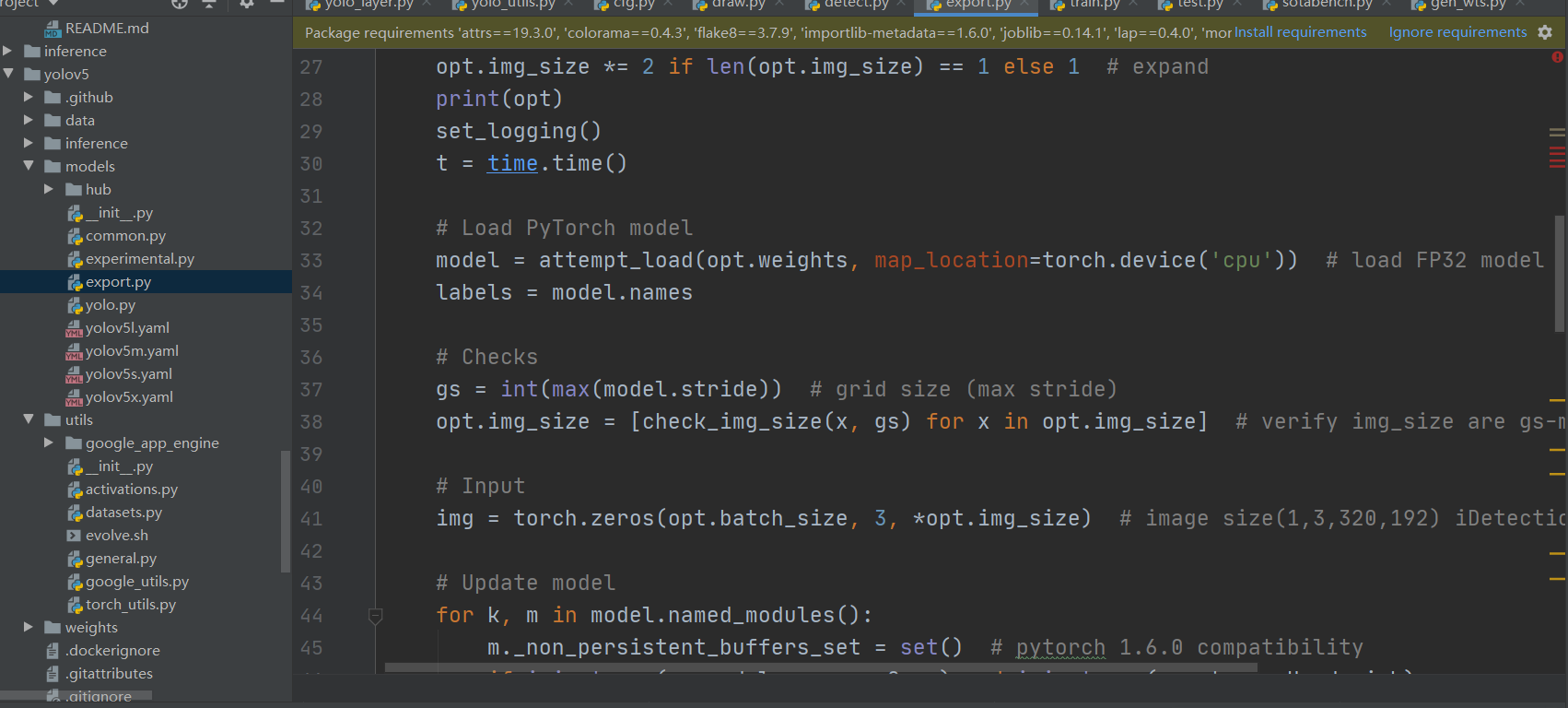## 演示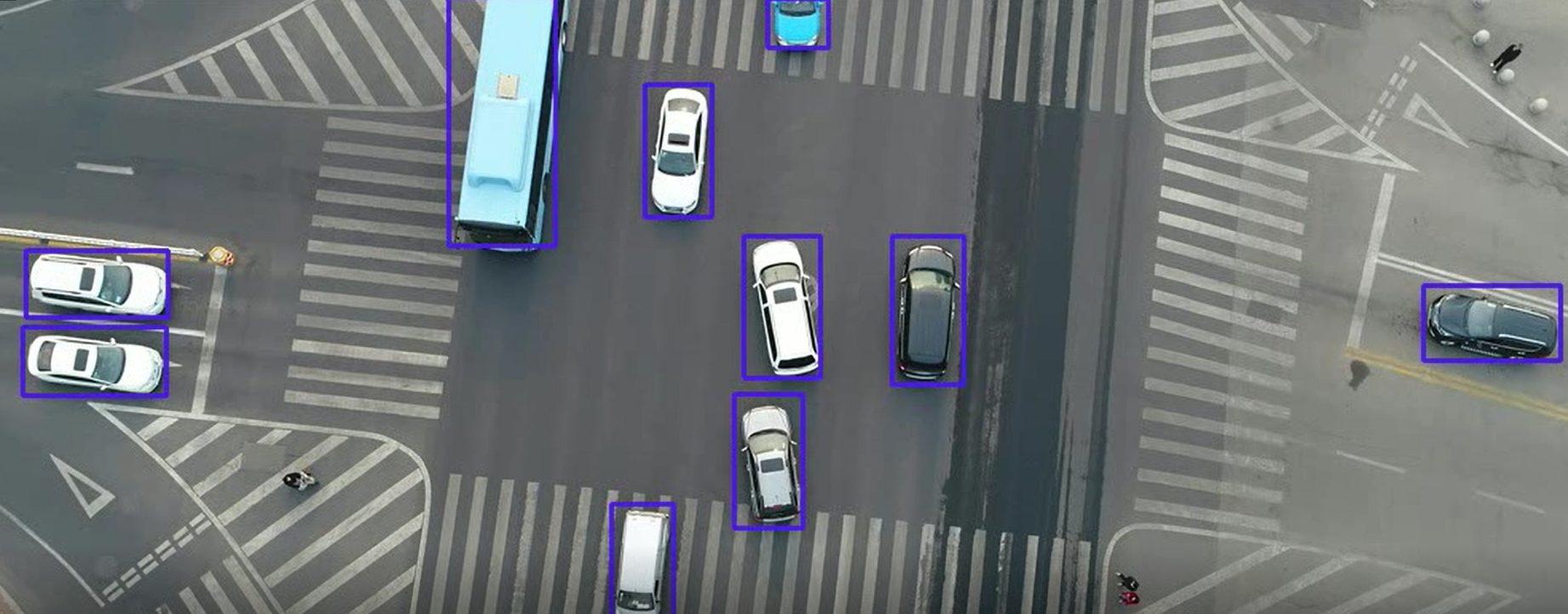## 总结## 评论 (1 条评论)2021 年 05 月 06 日 21:25